# Selina Solutions Concise Maths Class 10 Chapter 20 Cylinder, Cone and Sphere (Surface Area and Volume)

This chapter is one of the important chapters to concentrate on for ICSE Class 10.The key topics covered in this chapter are finding surface area and volume of cylinders, cones and spheres, conversion of solids, combination of solids and other miscellaneous problems. The Selina Solutions for Class 10 Mathematics prepared by our experienced faculty at BYJU’S, is created for the purpose of clarifying students’ doubts, as per their convenience. It also provides guidance to students for solving problems confidently. Thus, improving their problem-solving skills, which are vital from an examination point of view. The Selina Solutions for Class 10 Mathematics Chapter 20 Cylinder, Cone and Sphere (Surface Area and Volume) PDF is available in the link below.

## Selina Solutions Concise Maths Class 10 Chapter 20 Cylinder, Cone and Sphere (Surface Area and Volume) Download PDF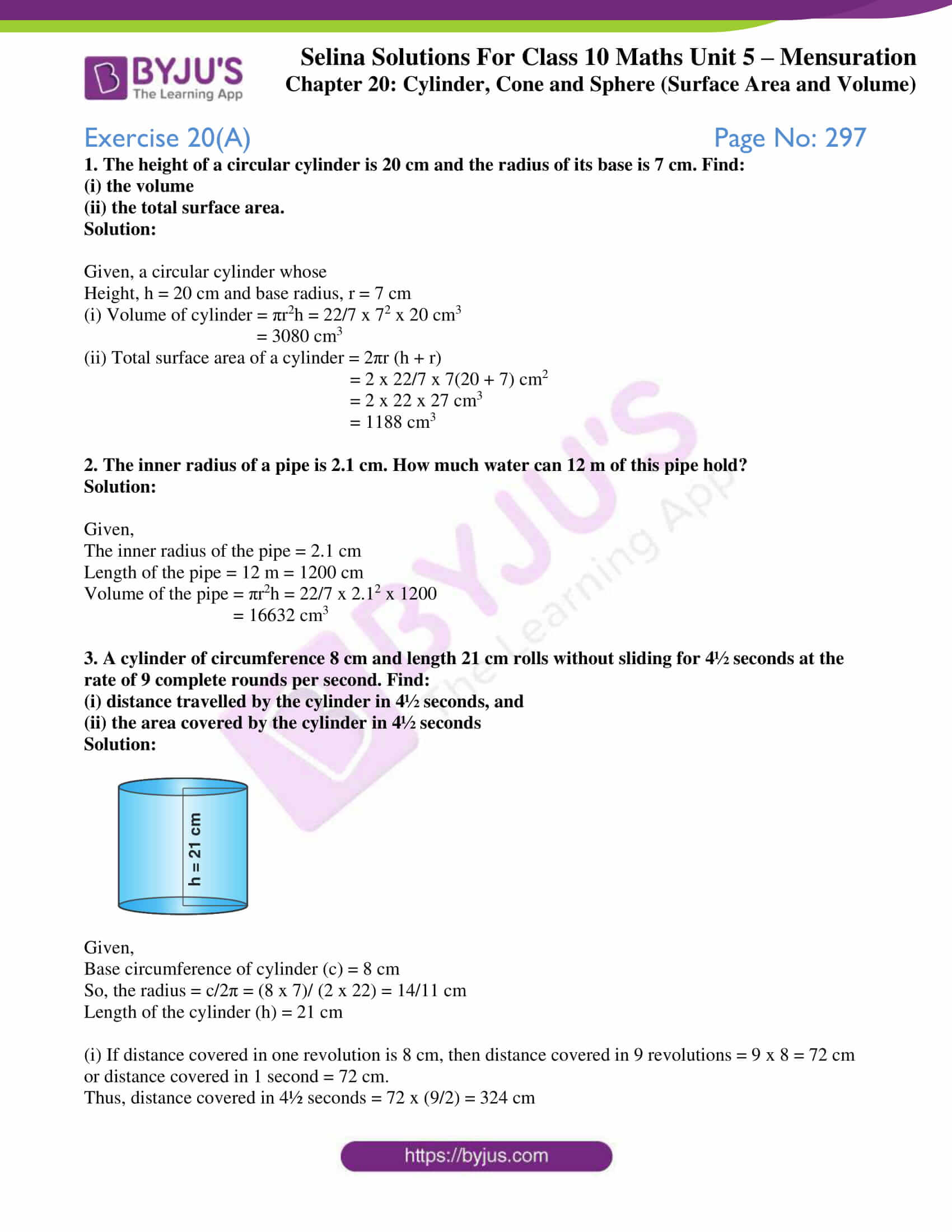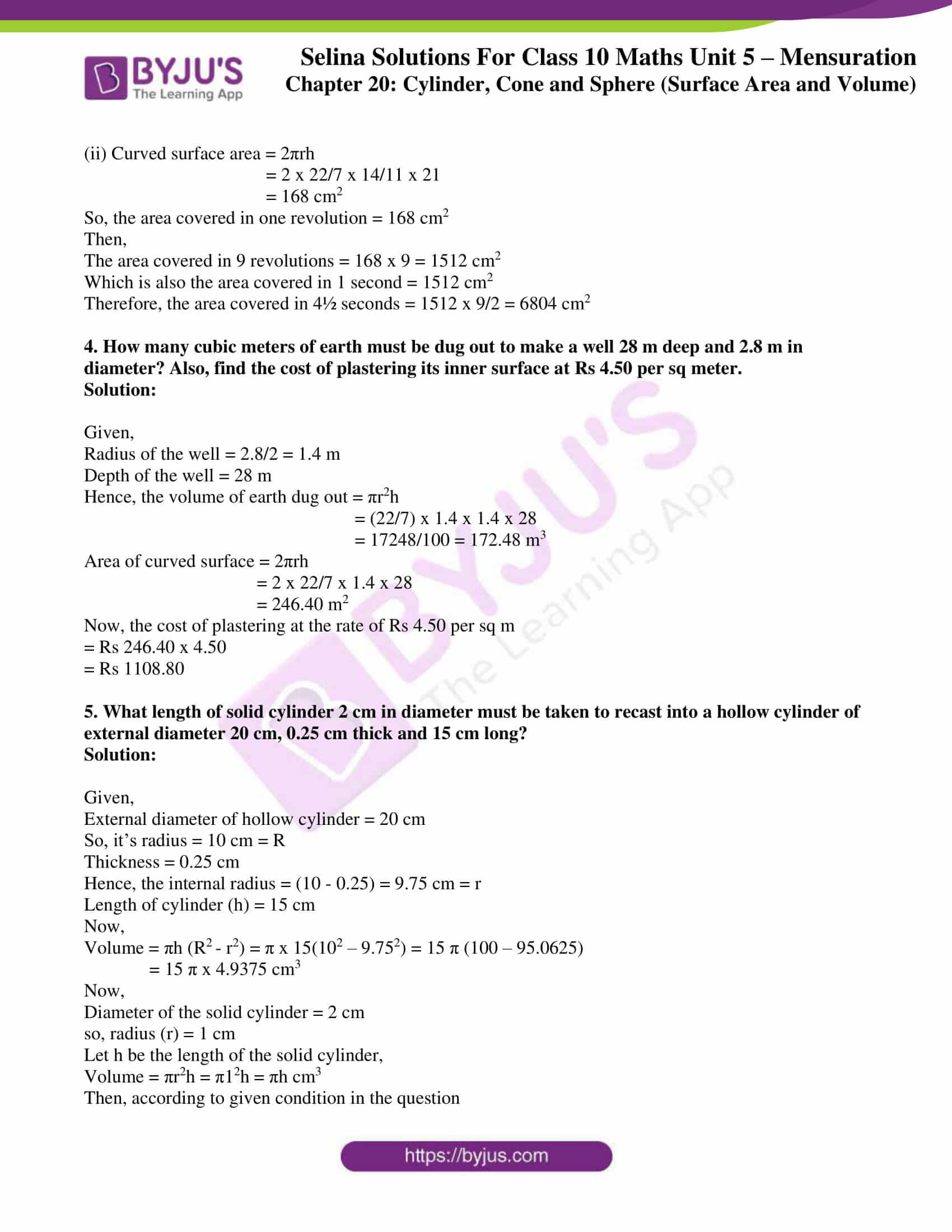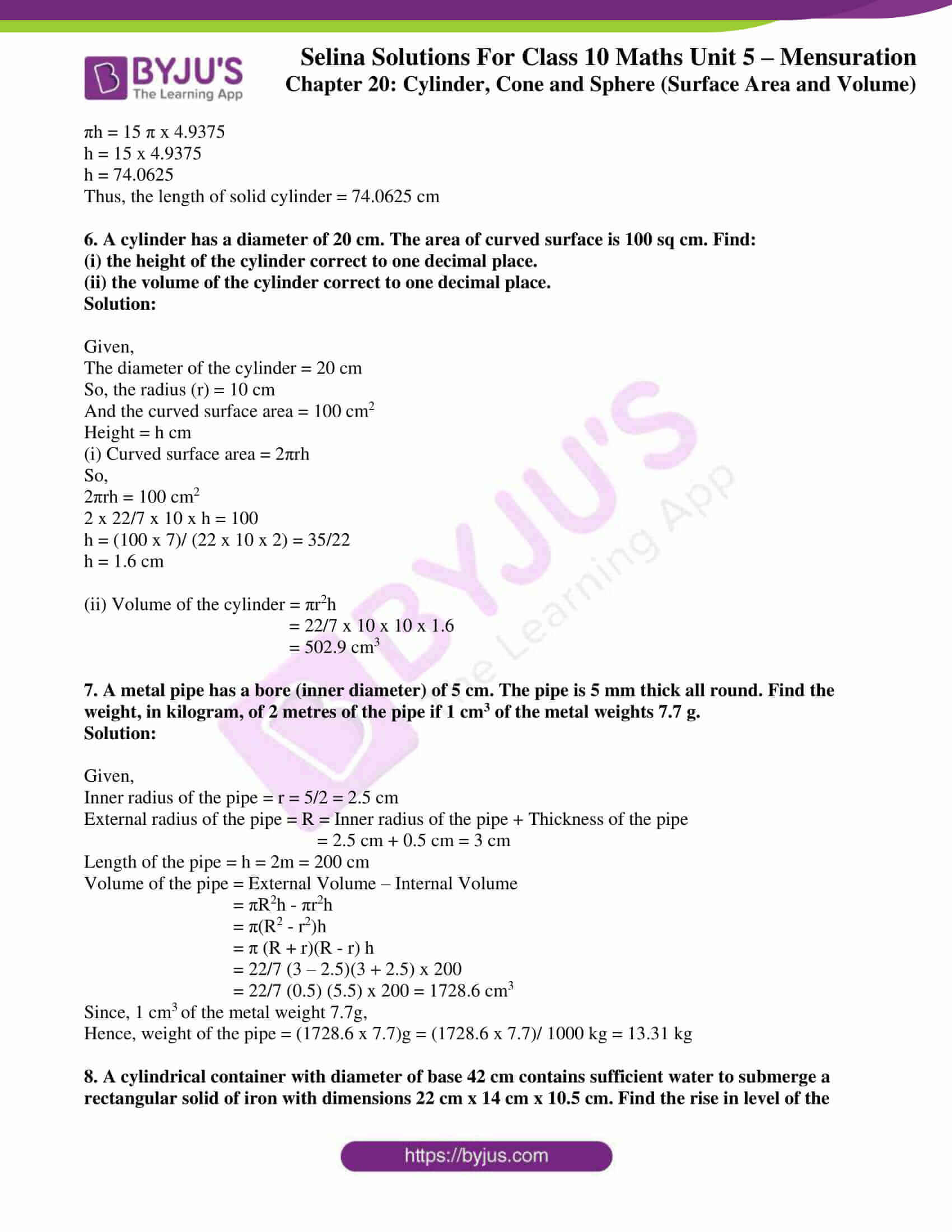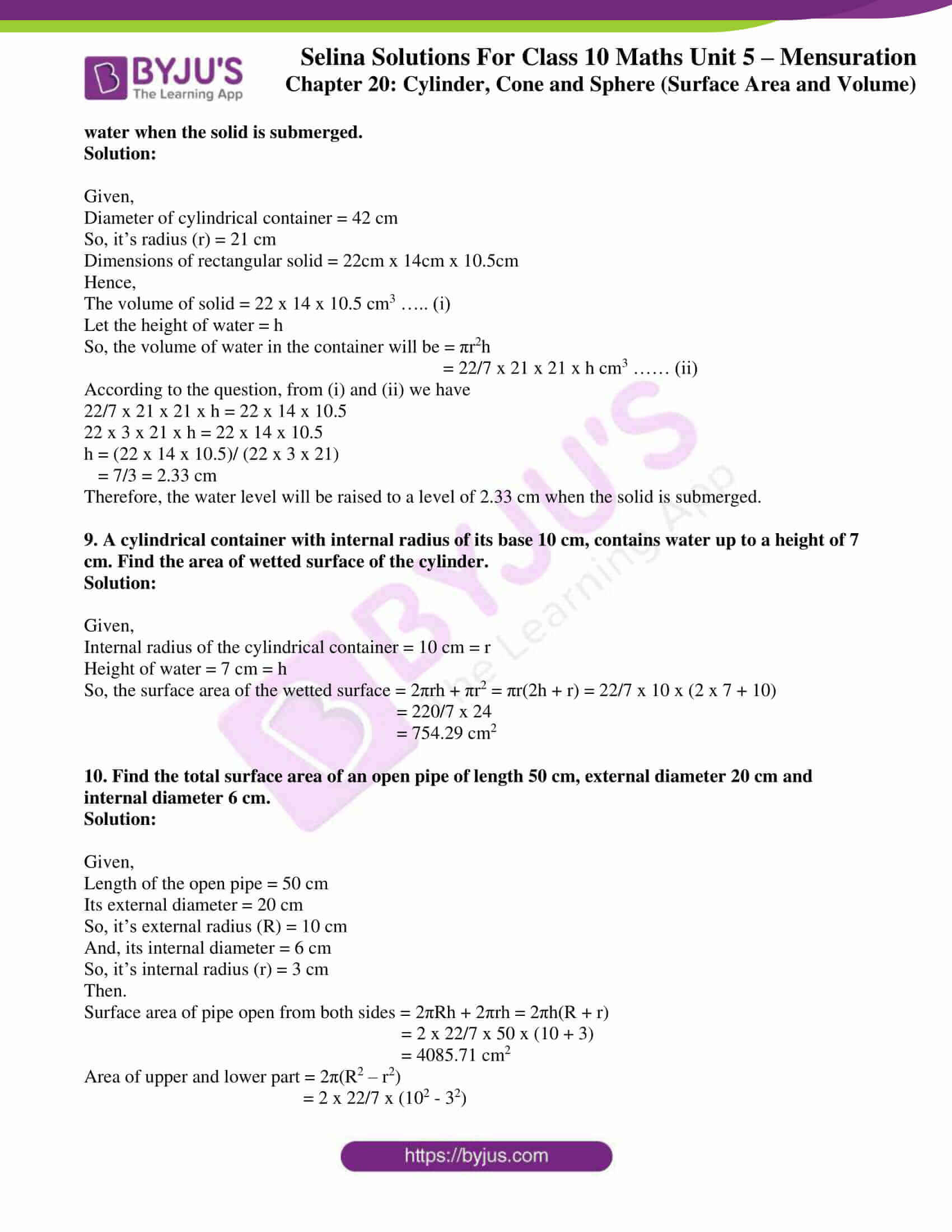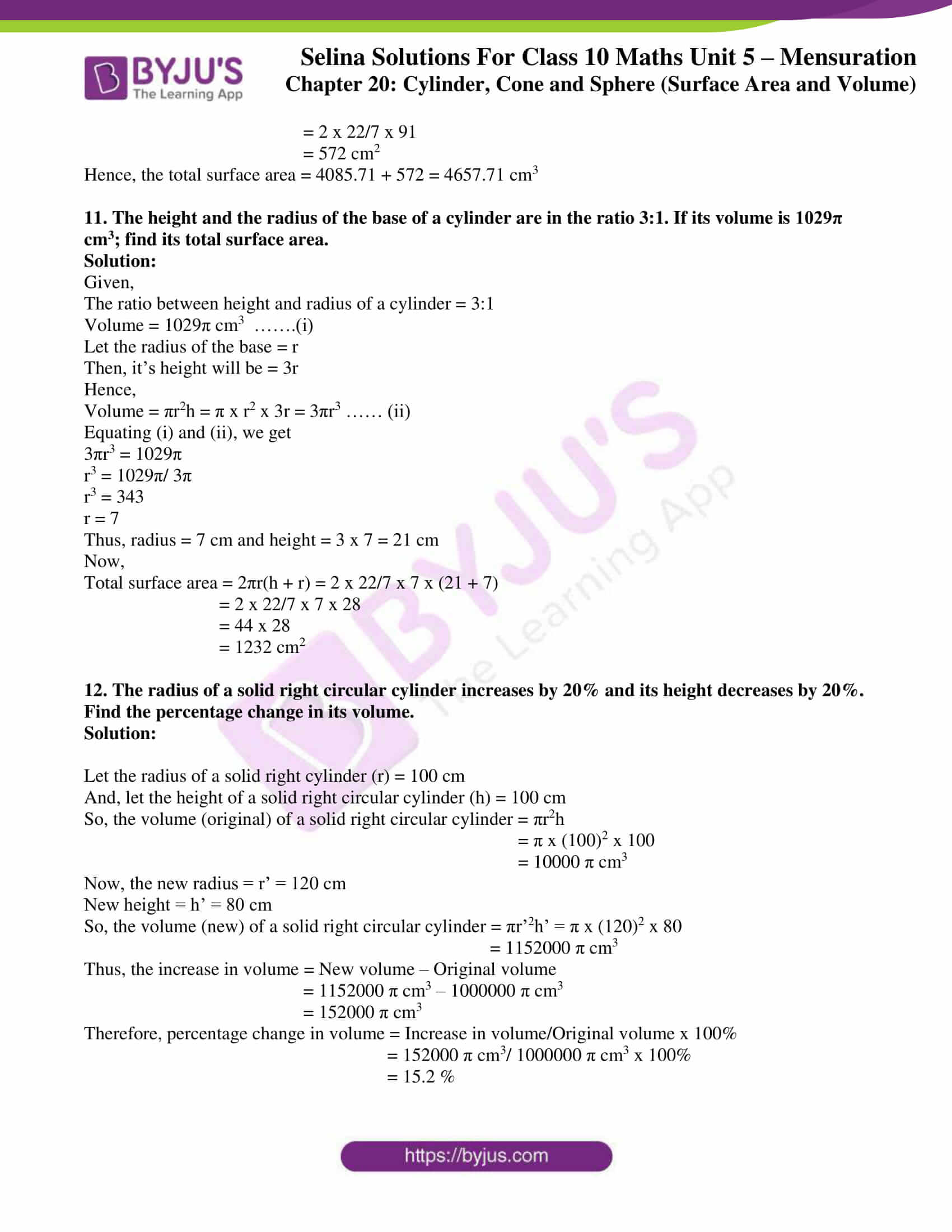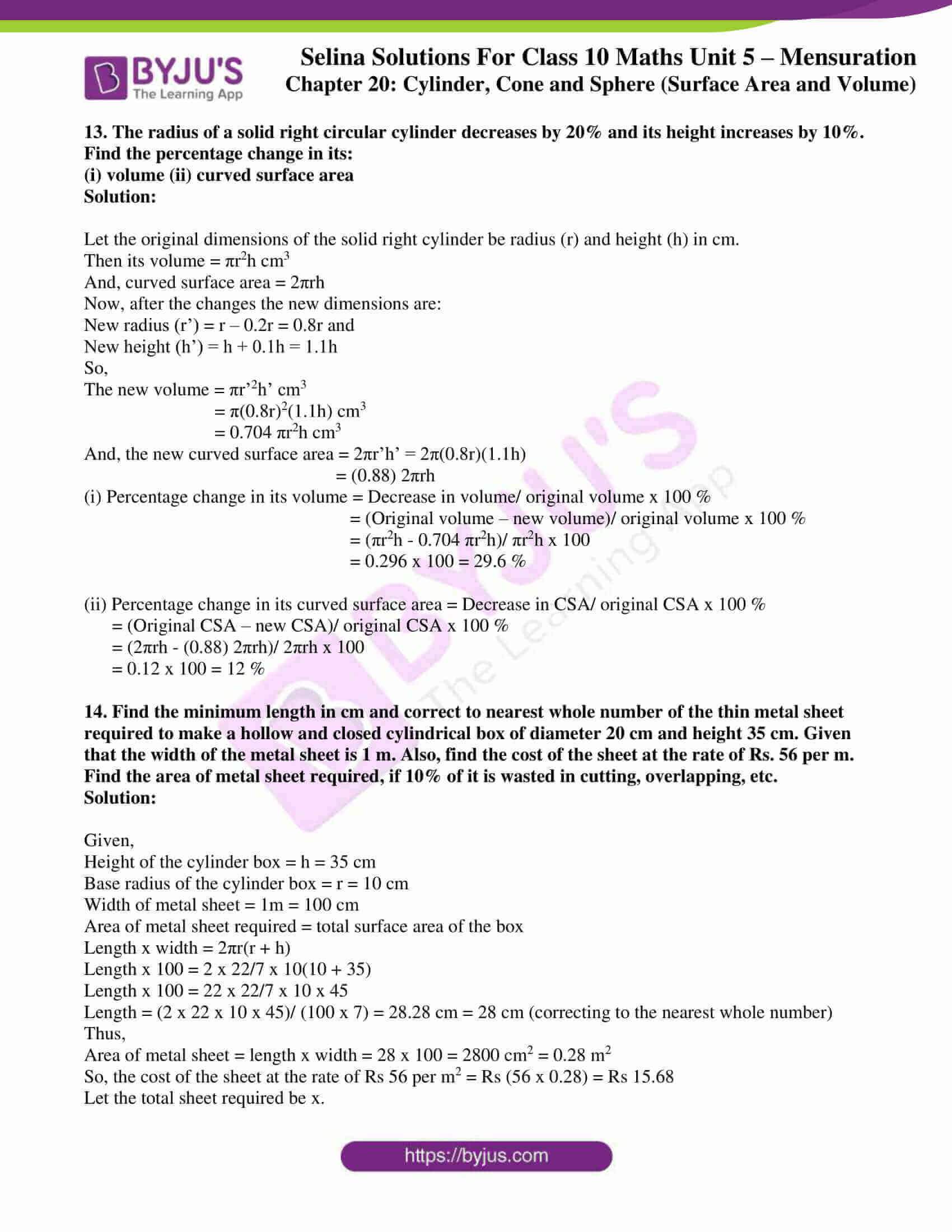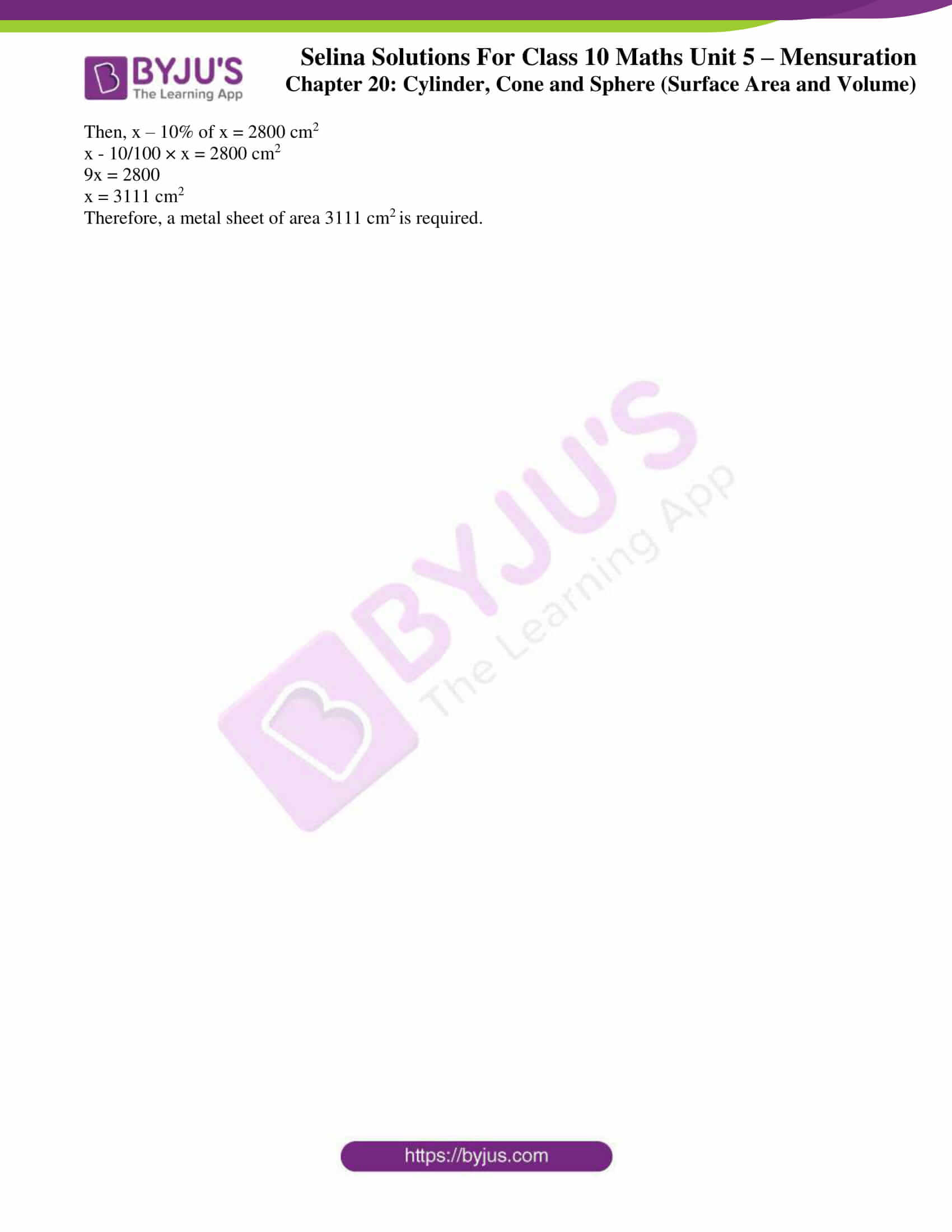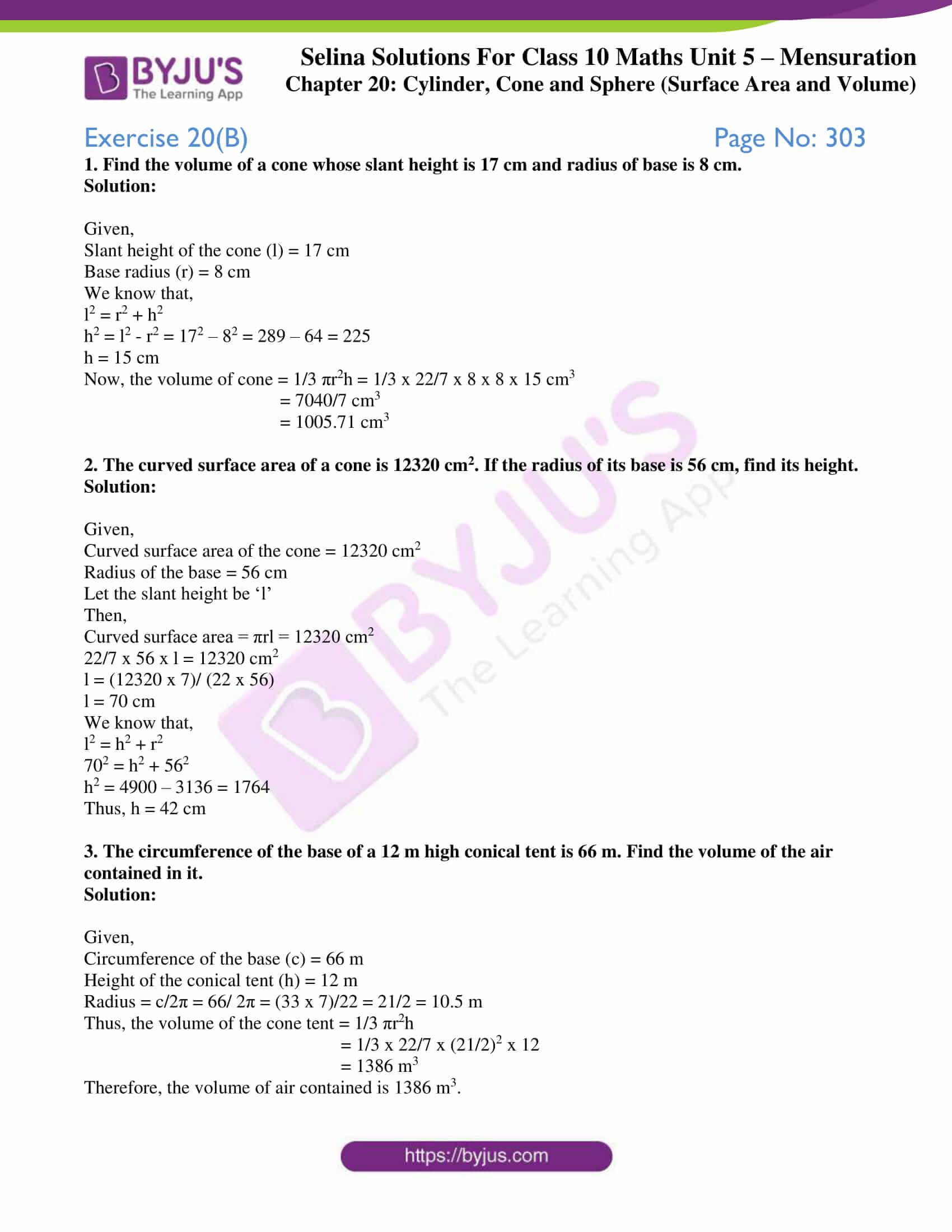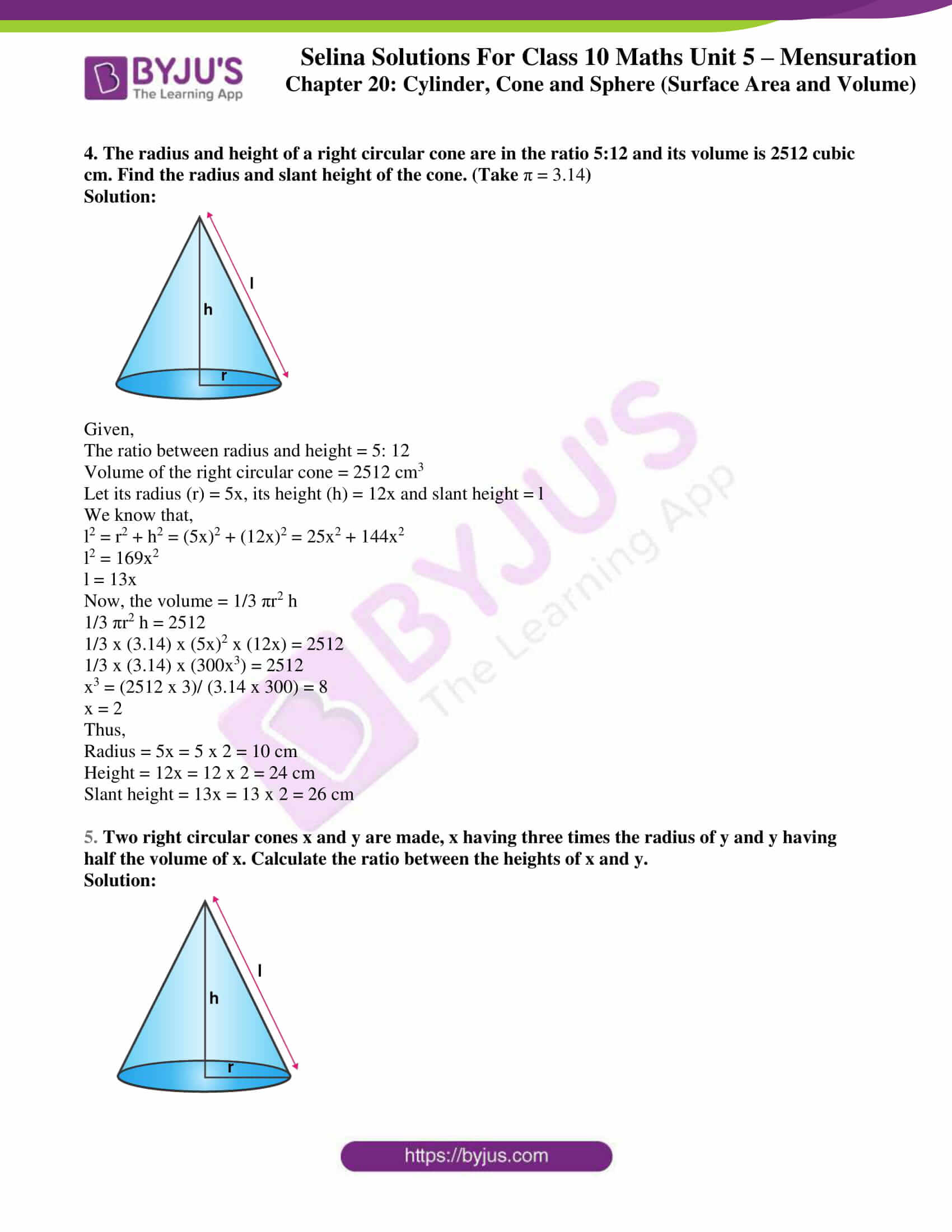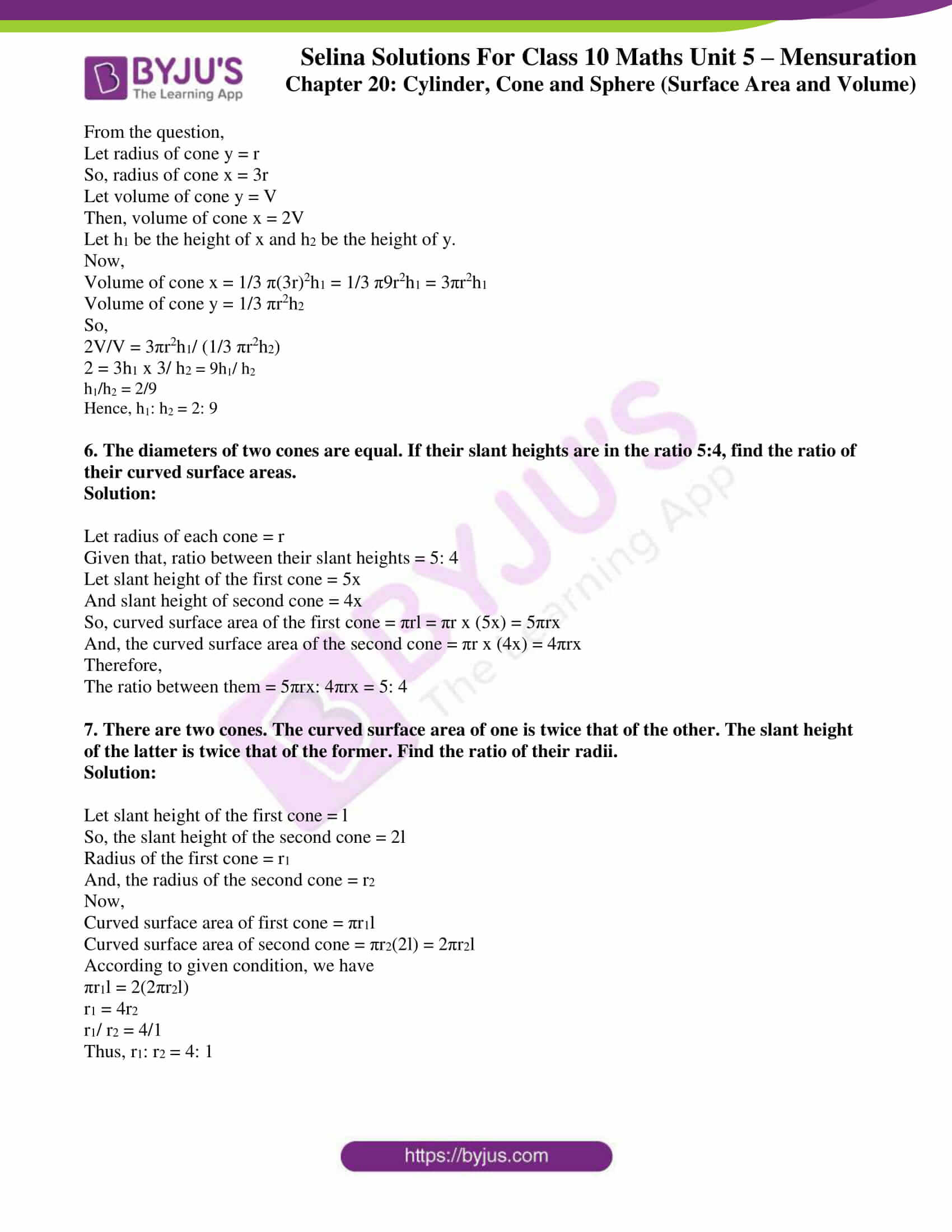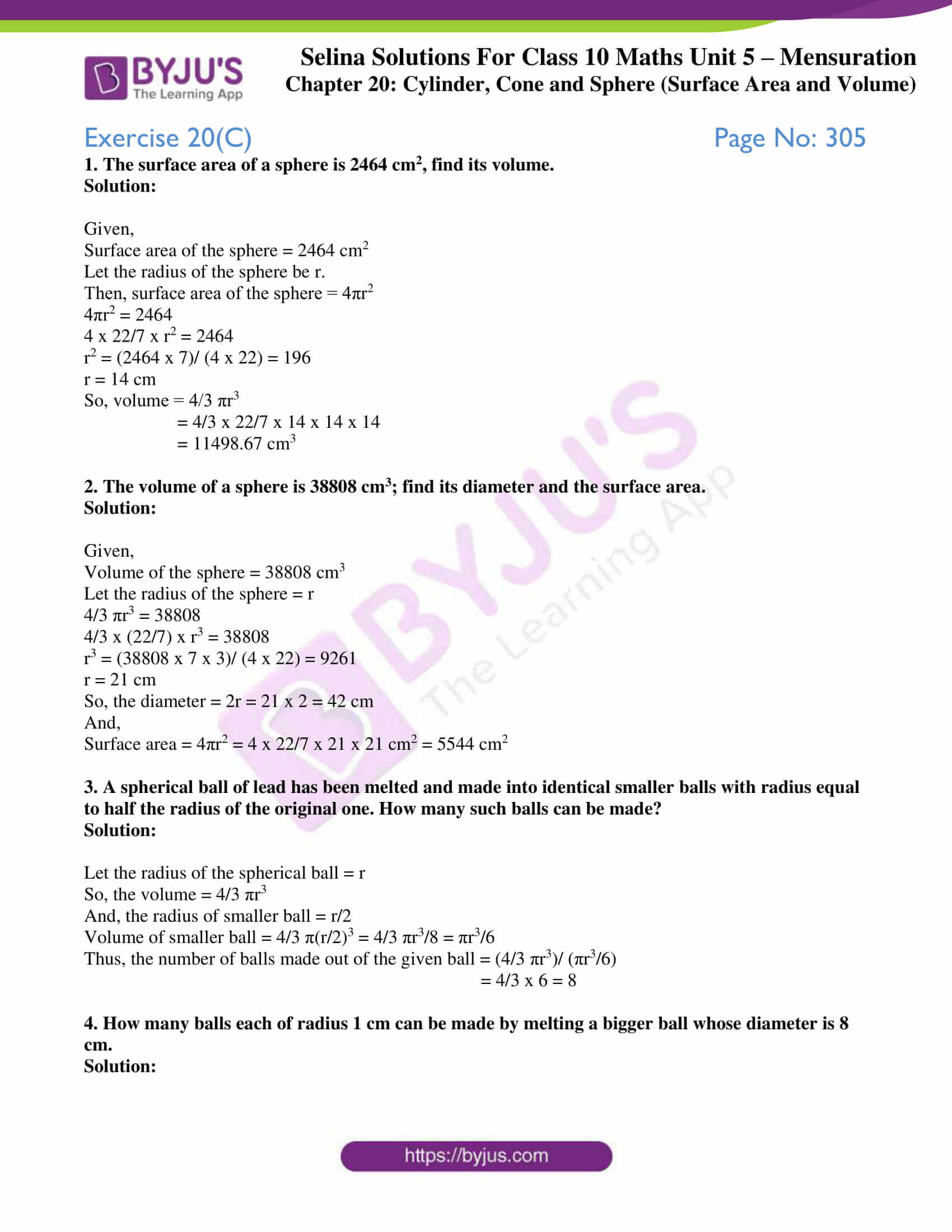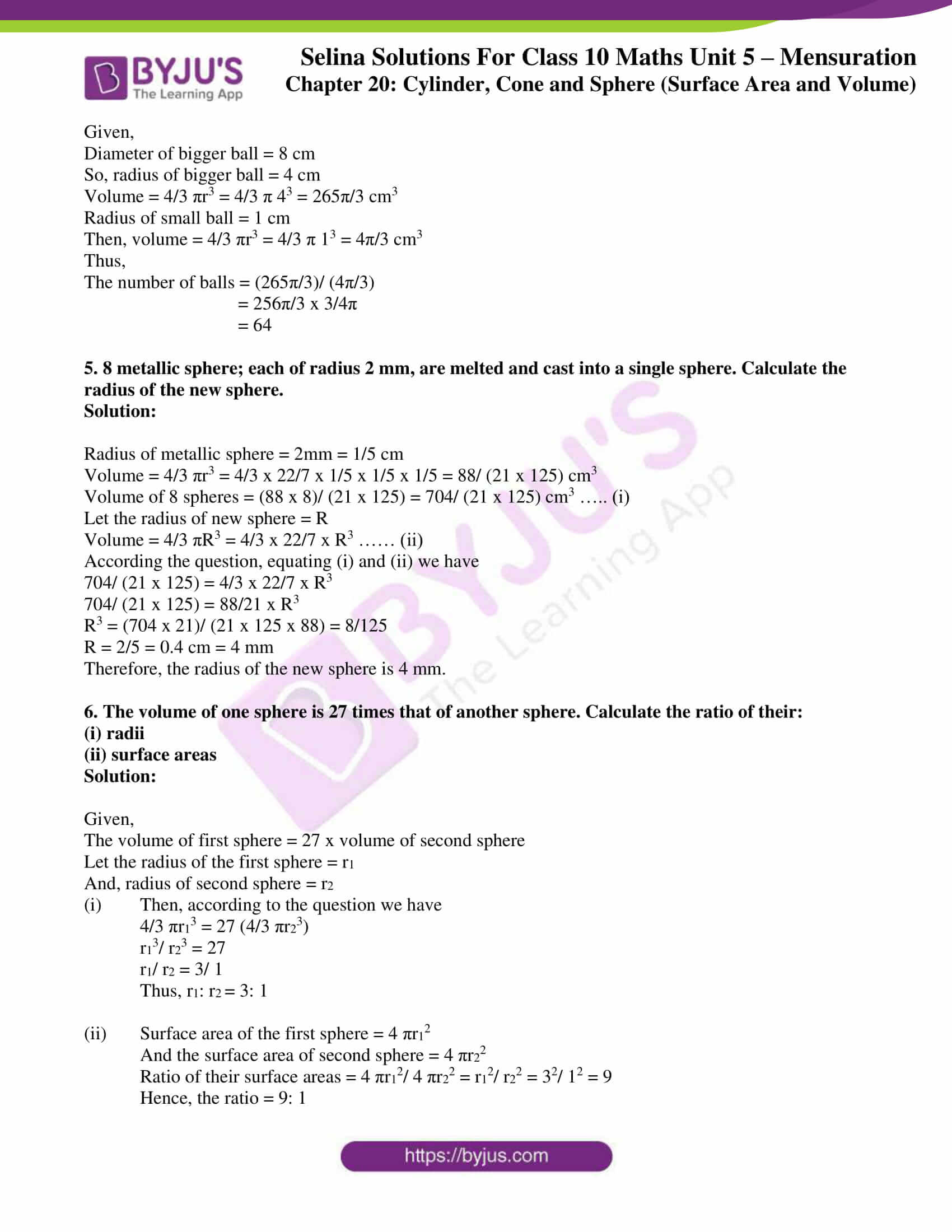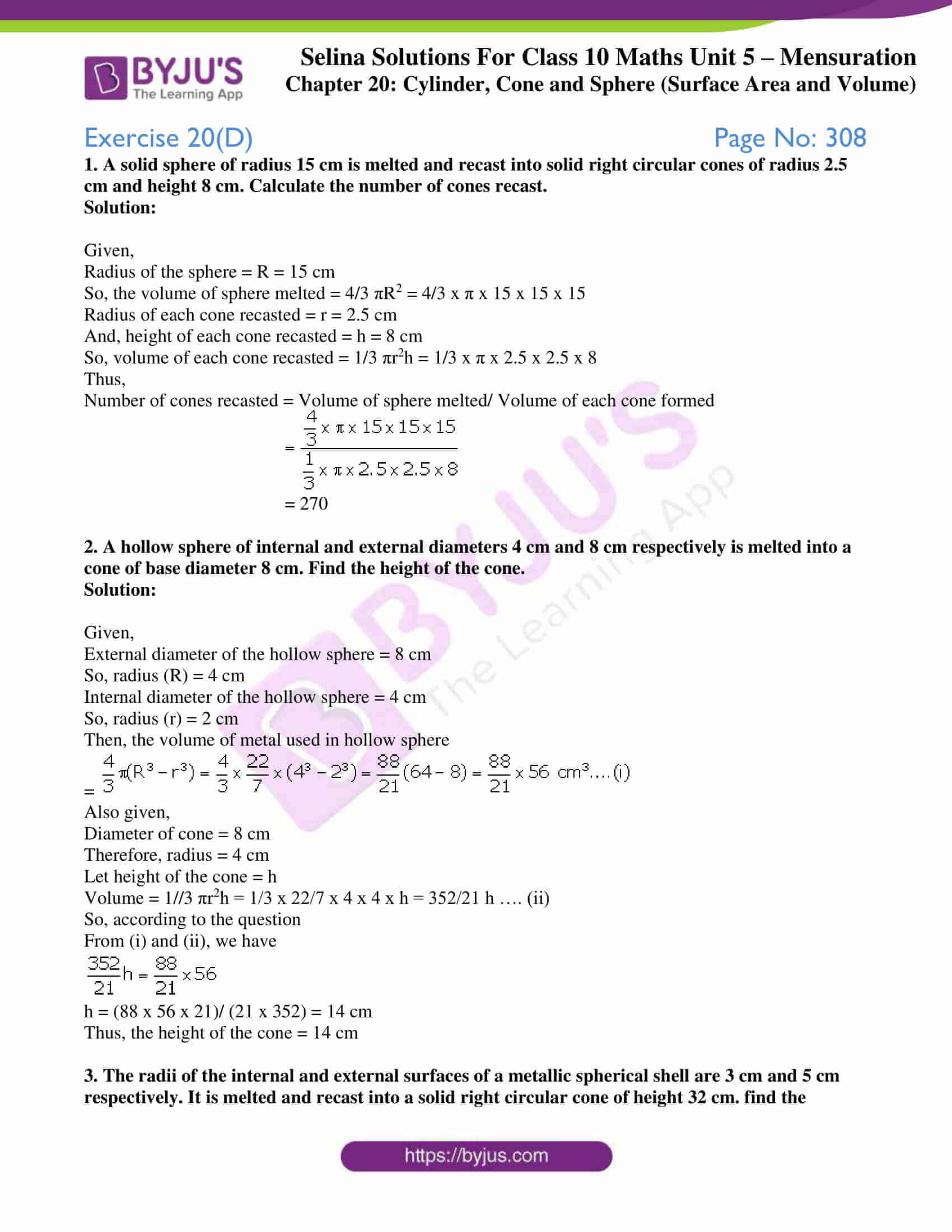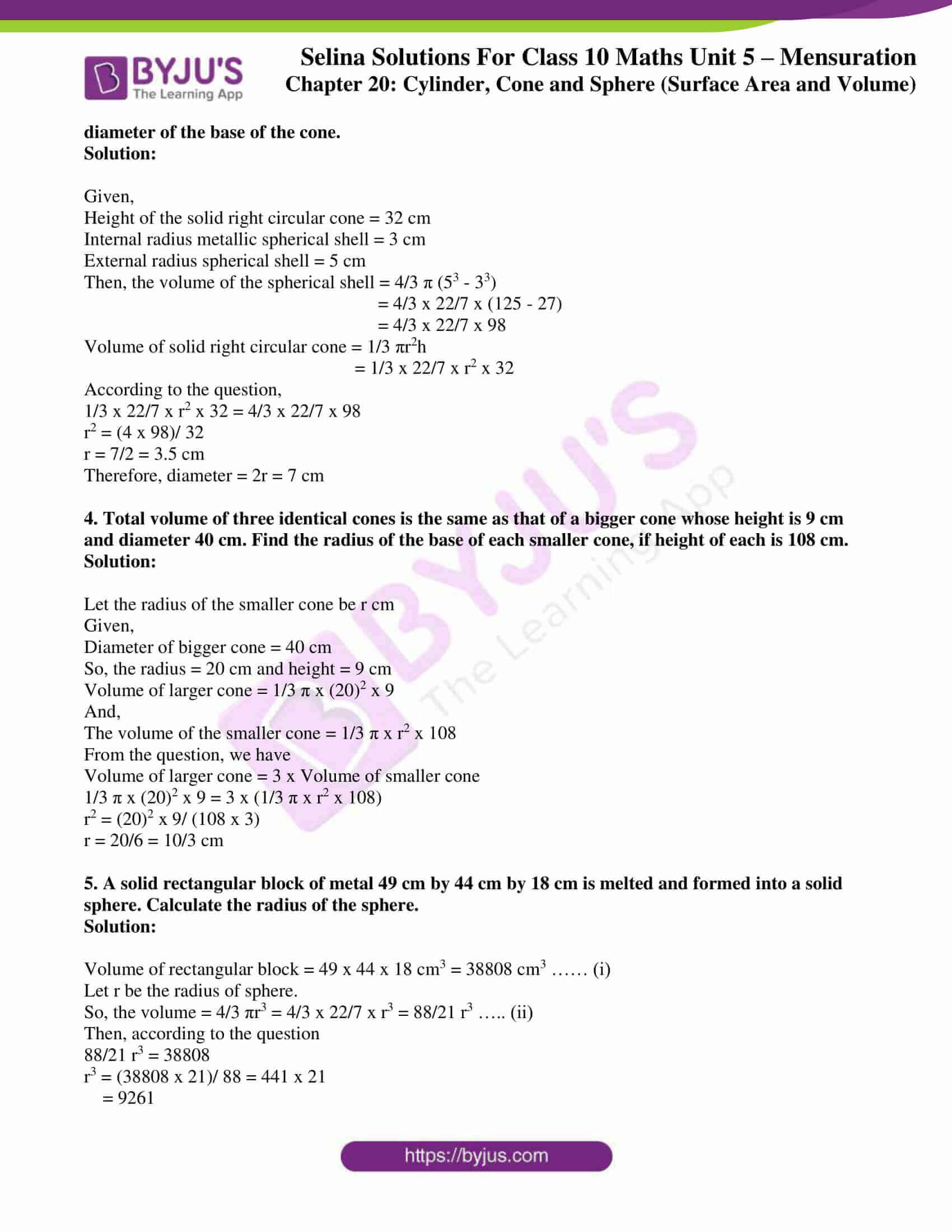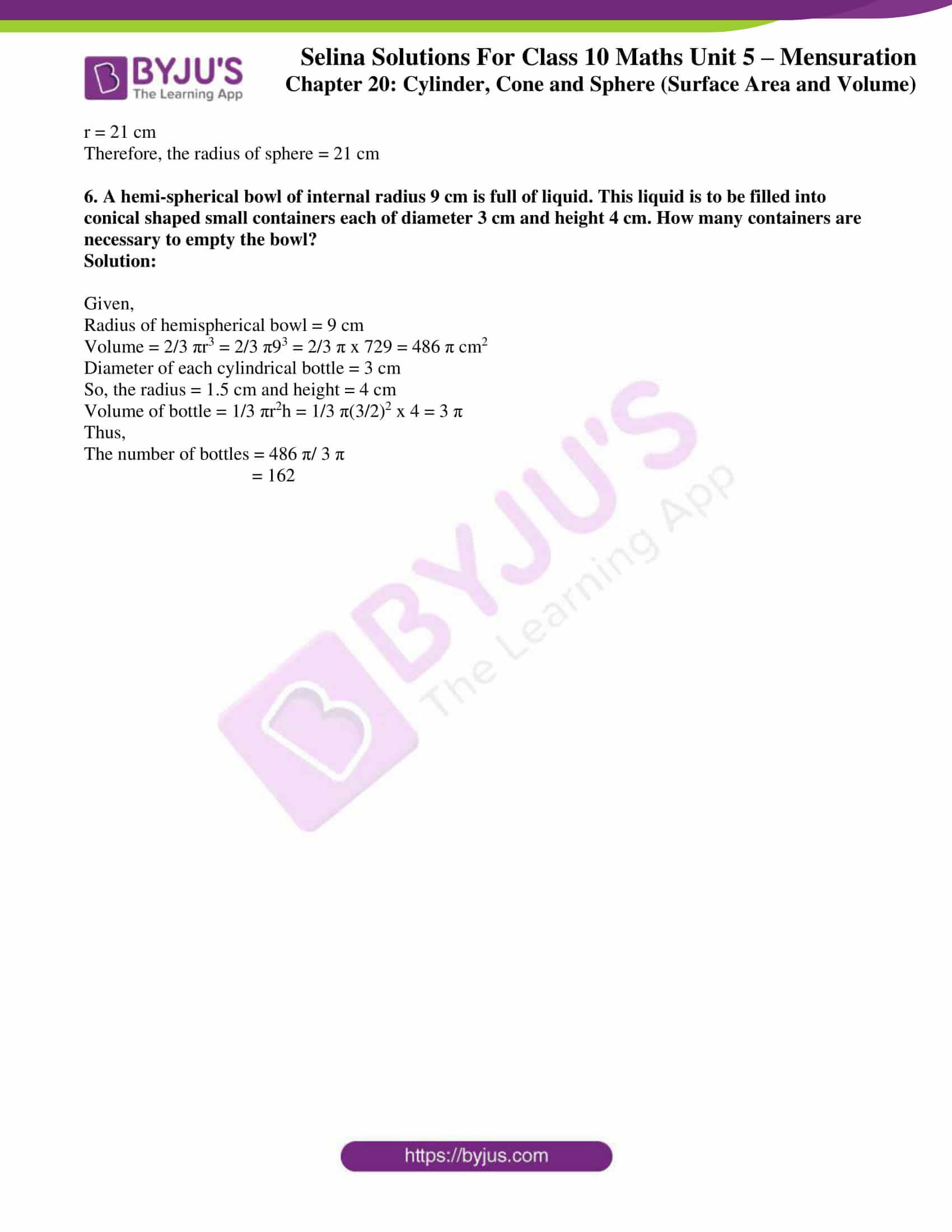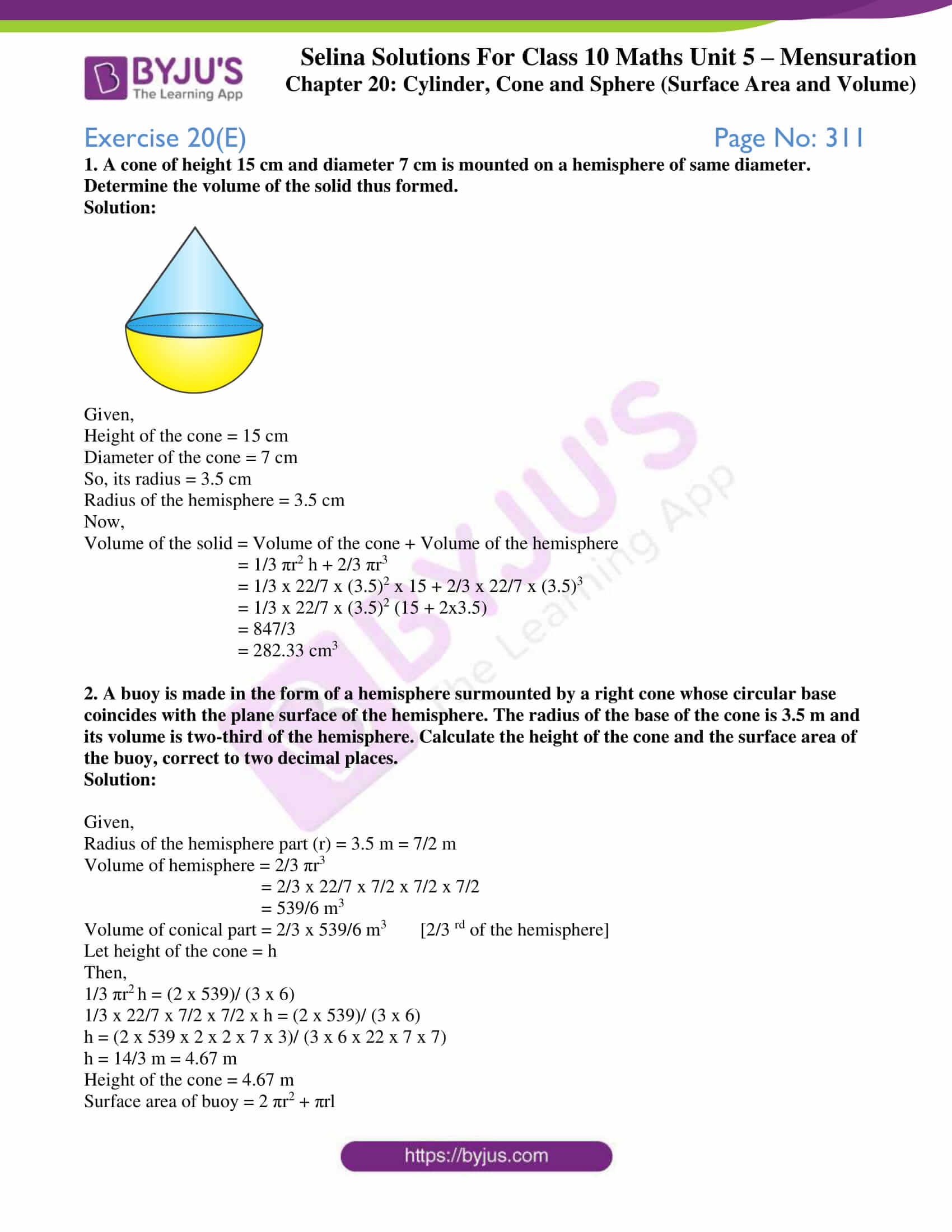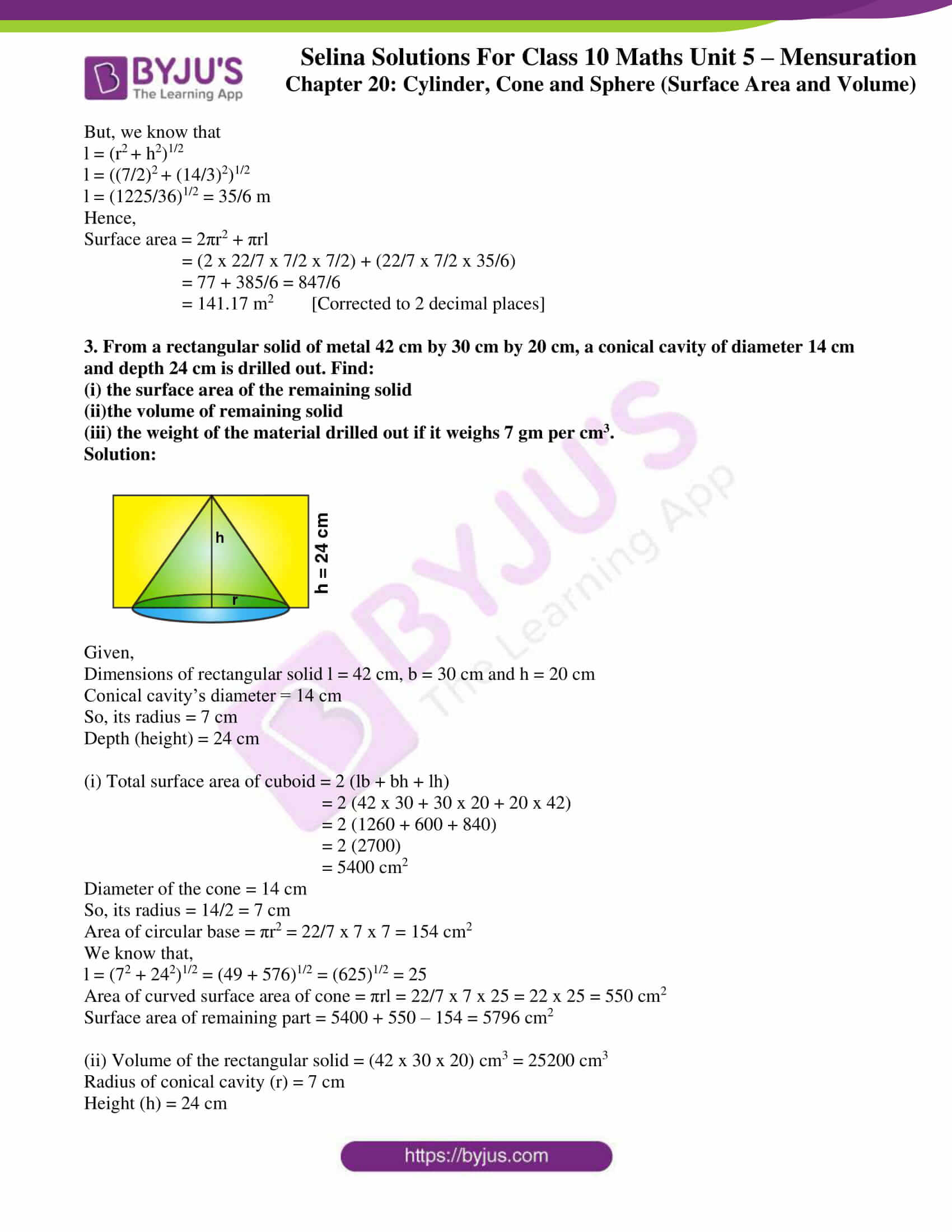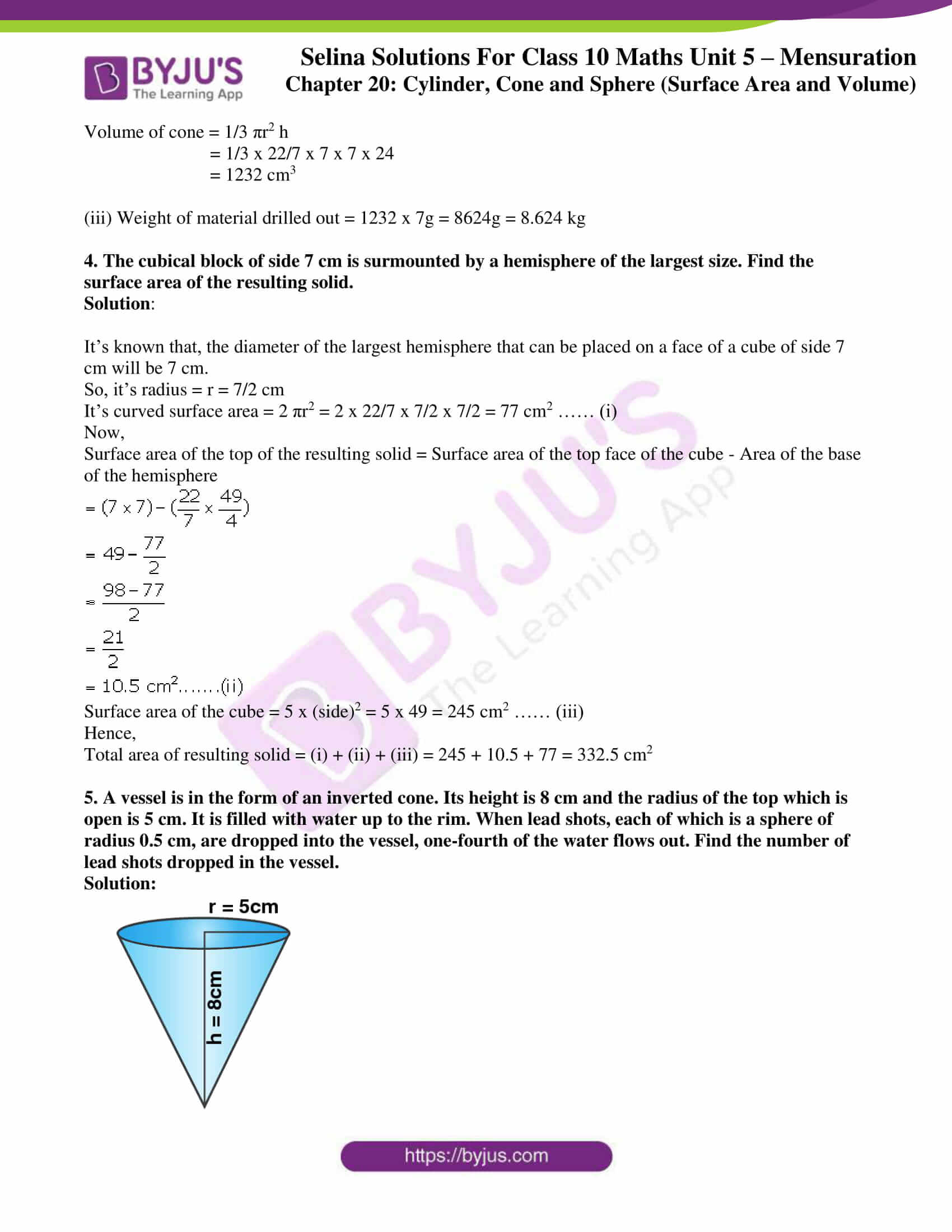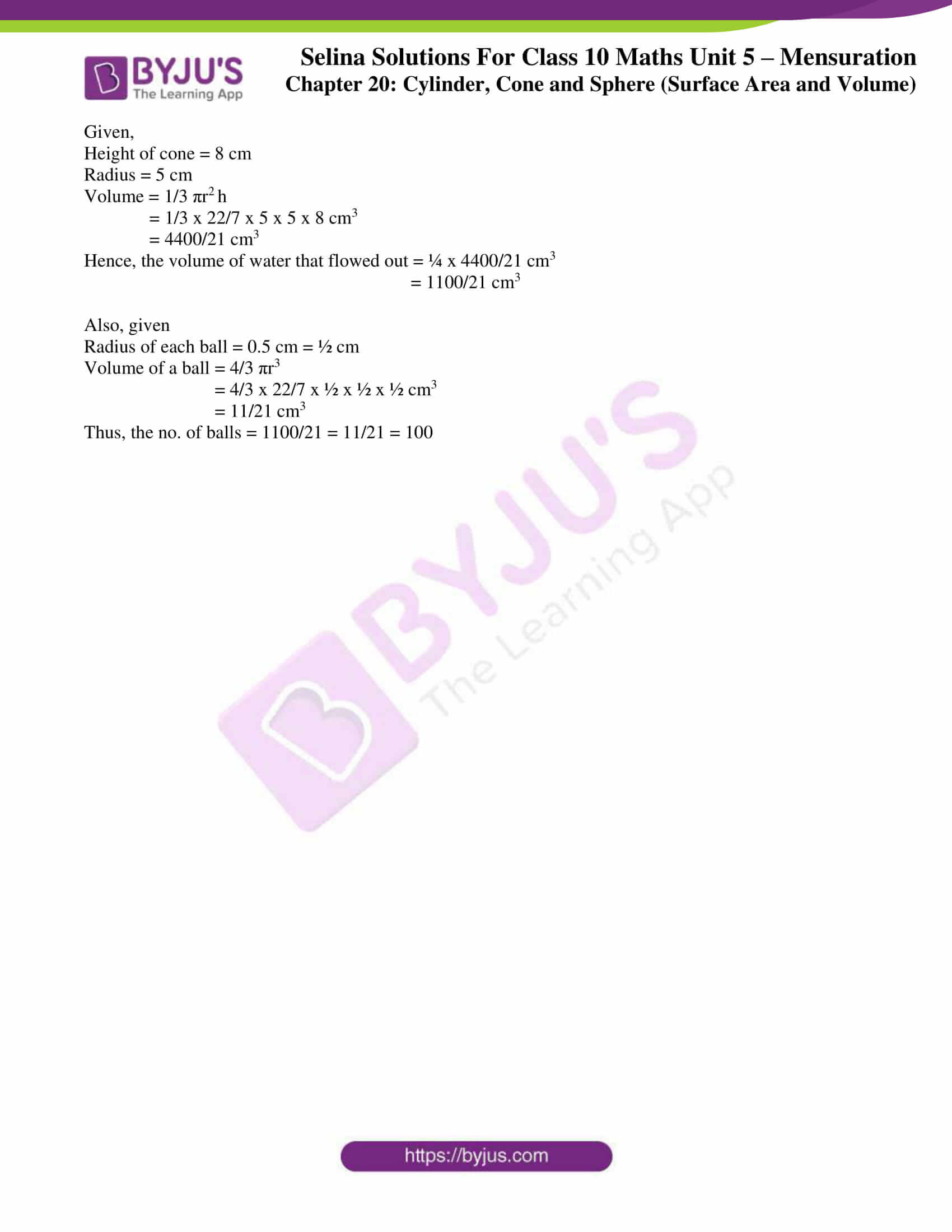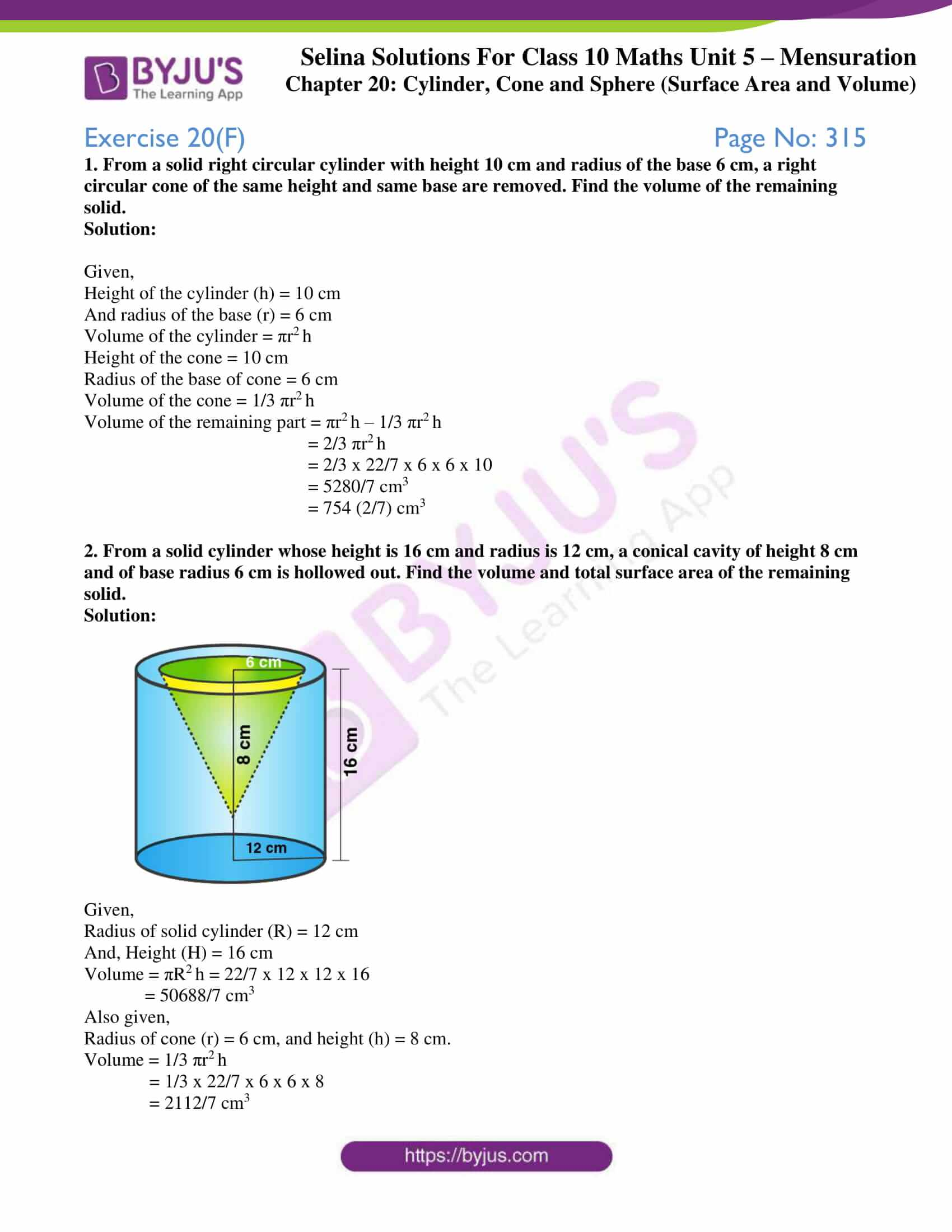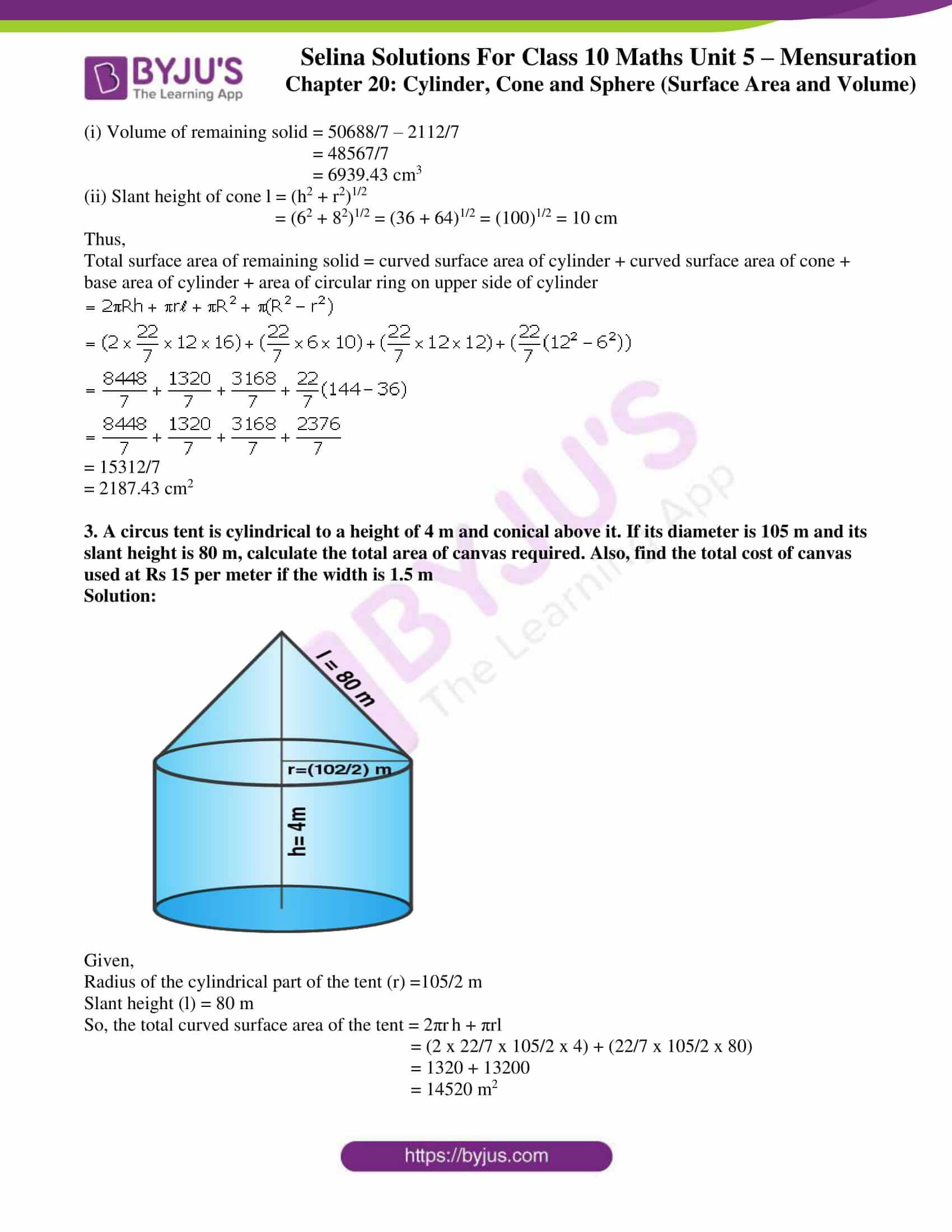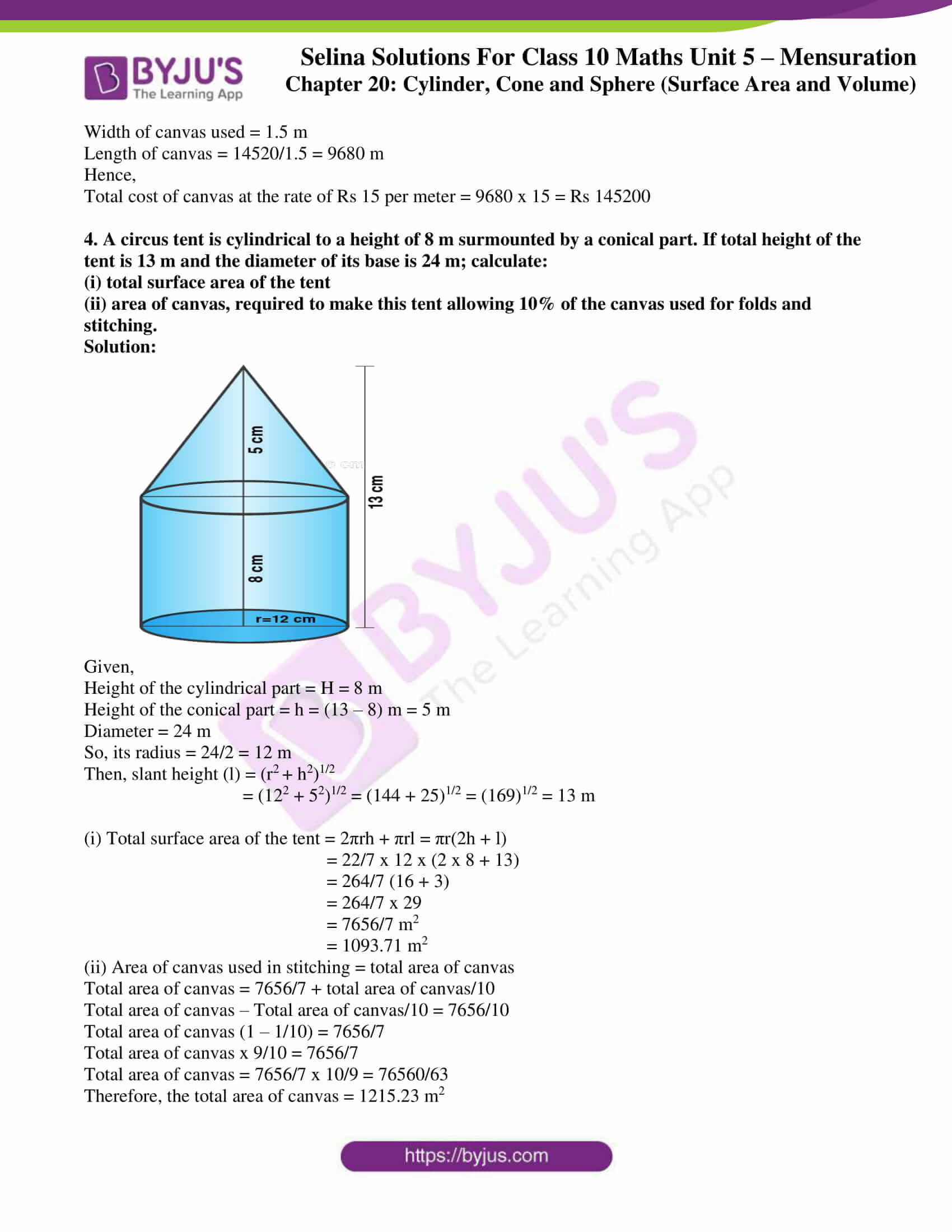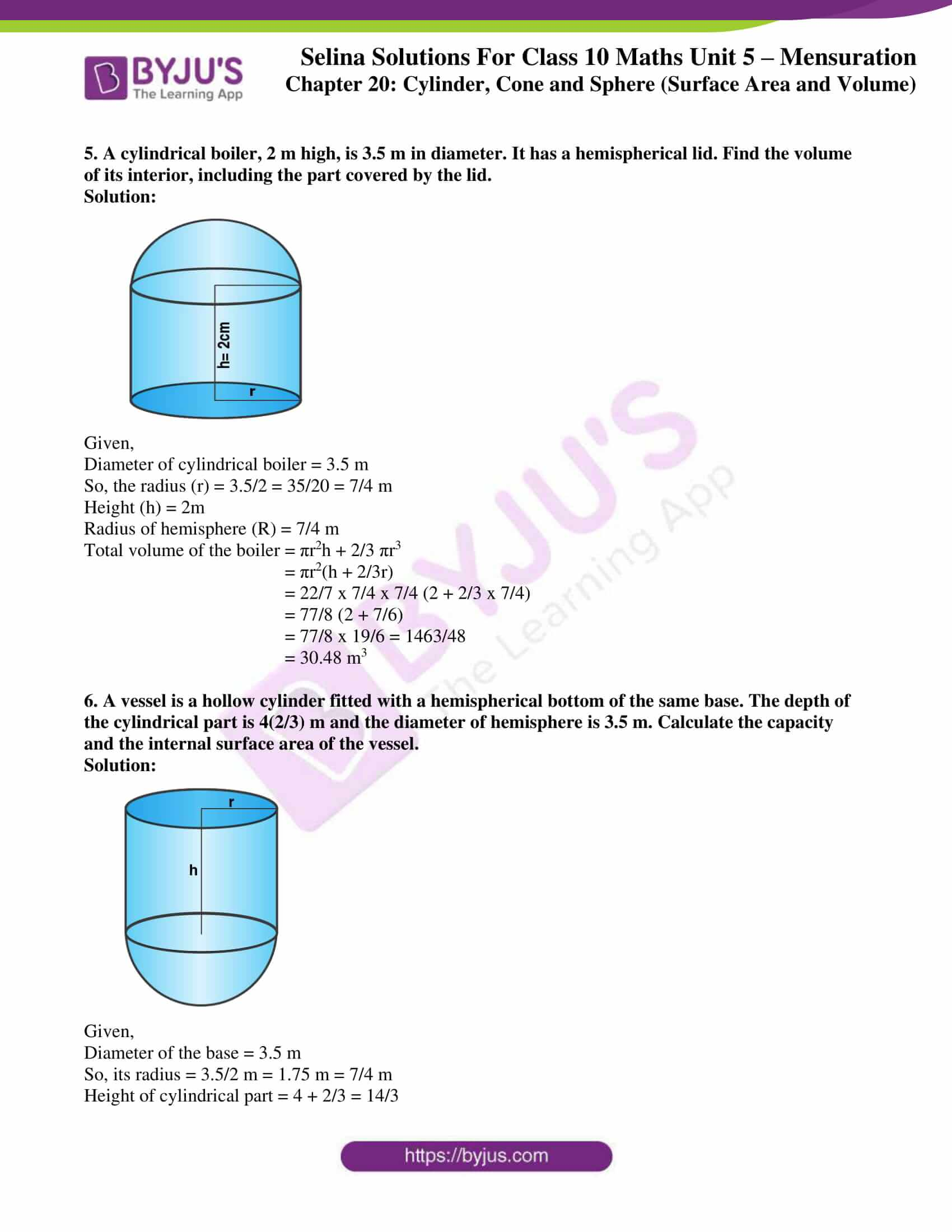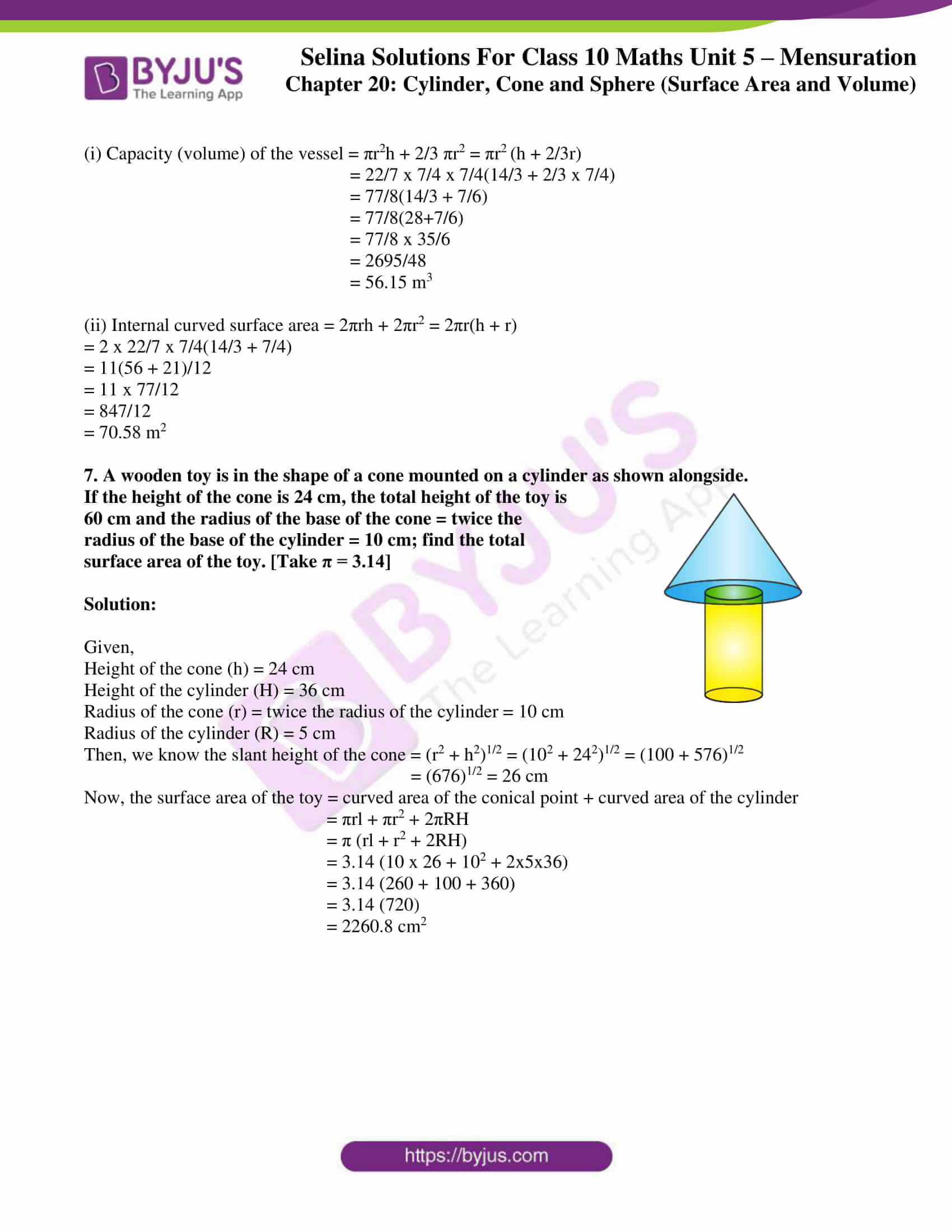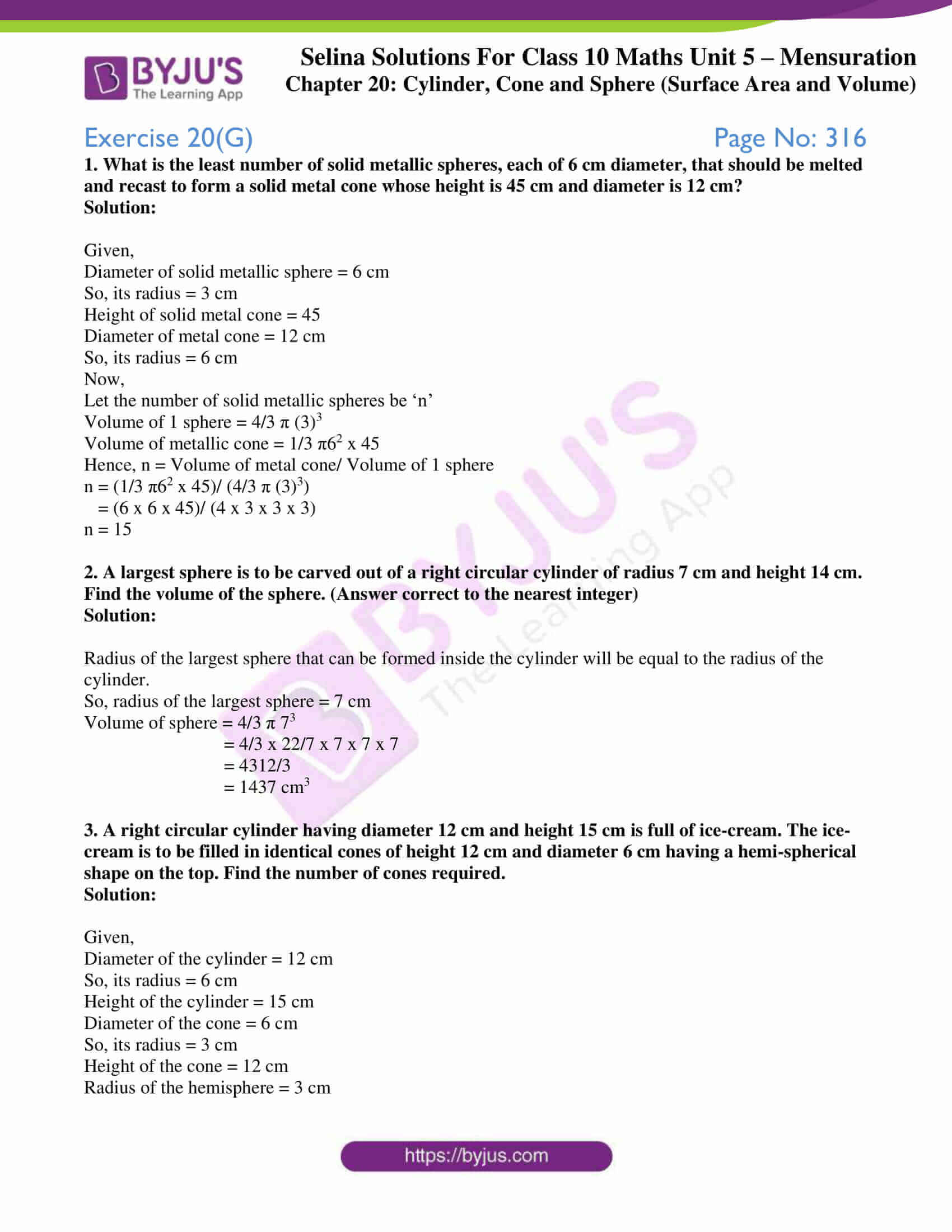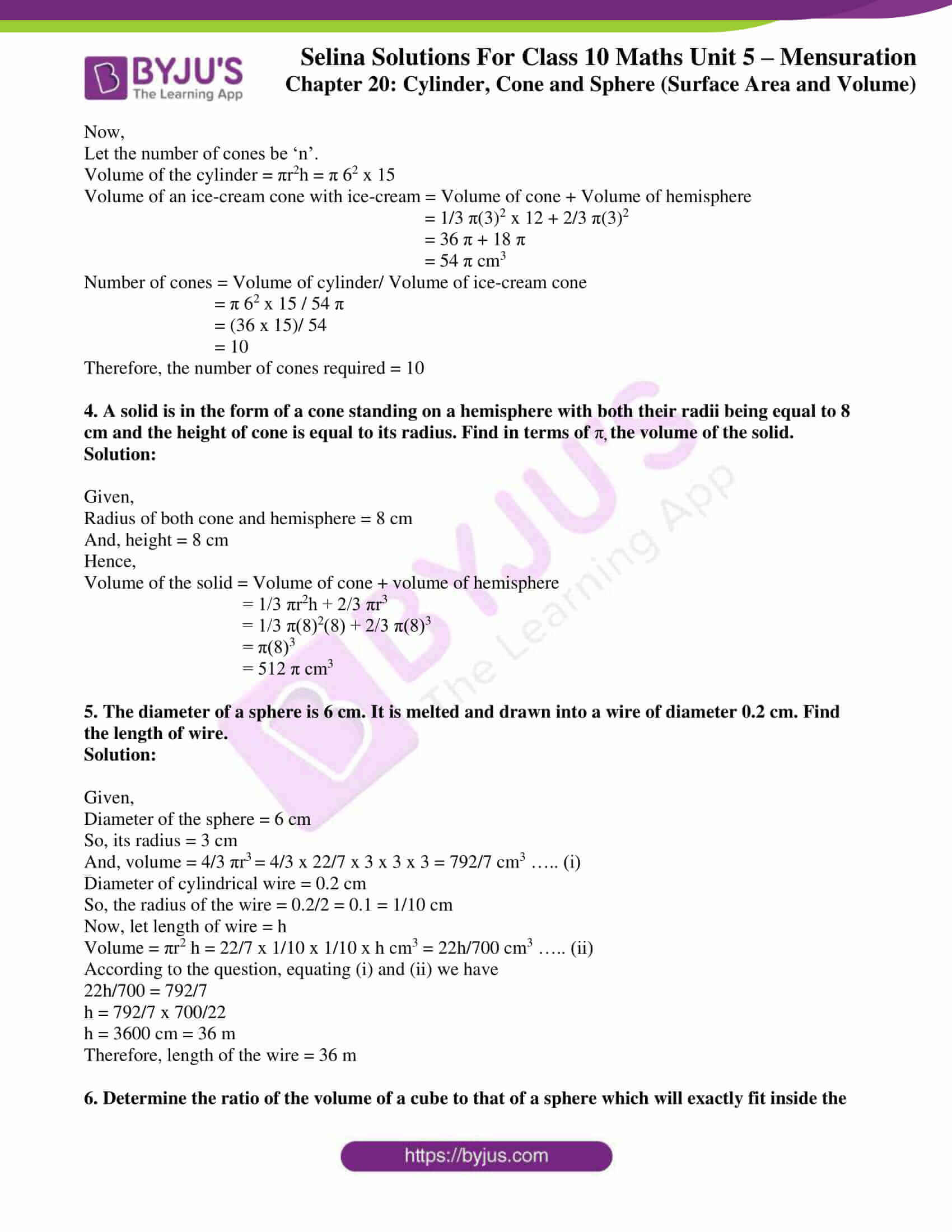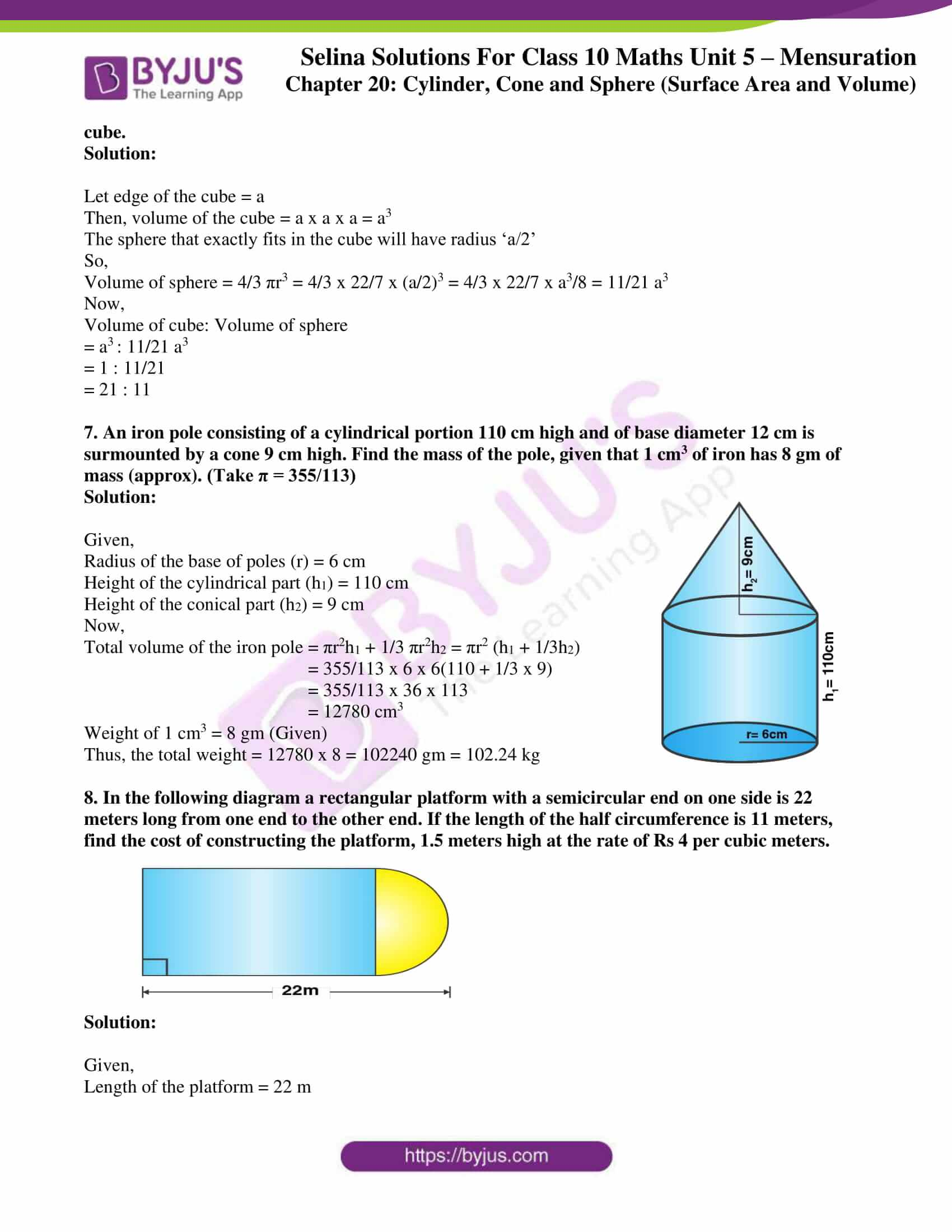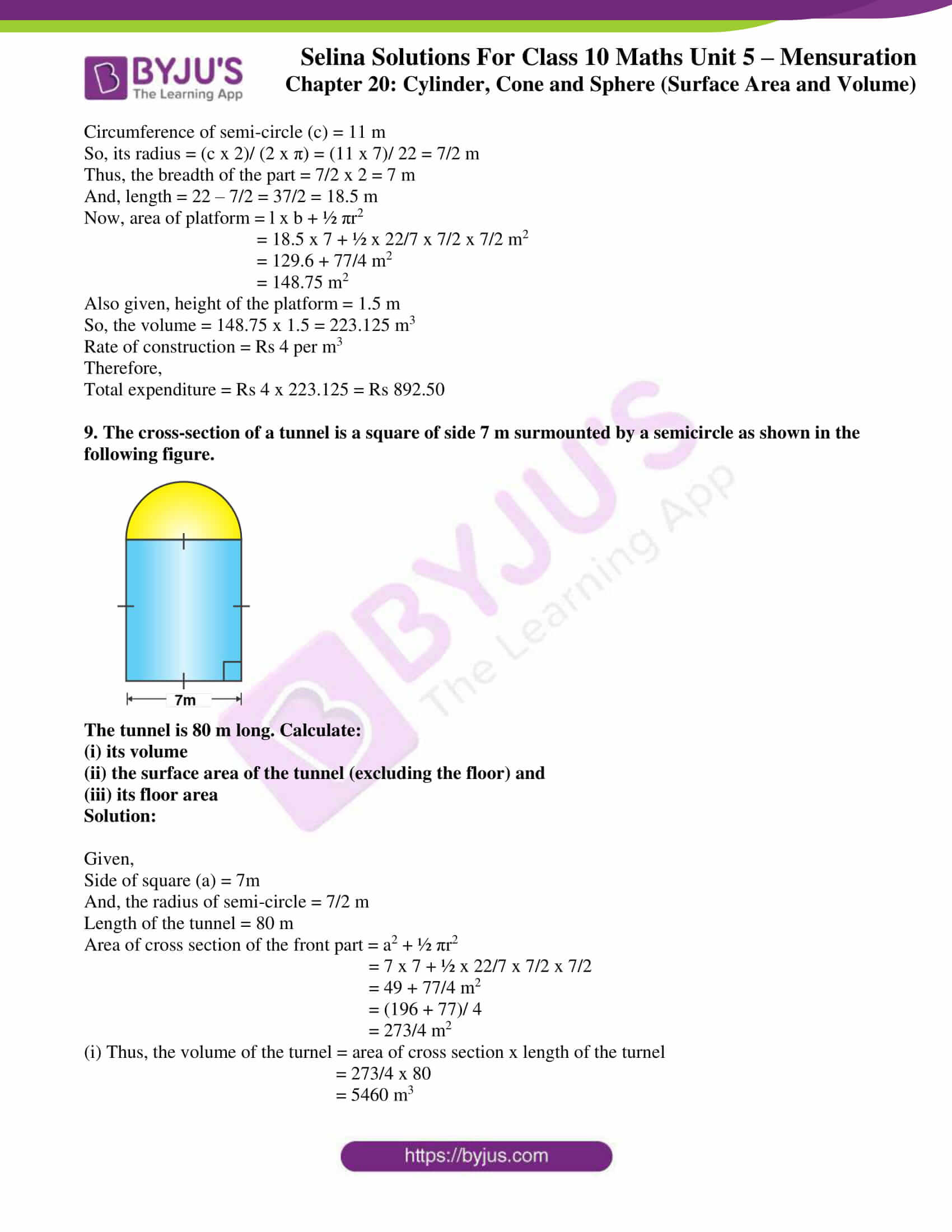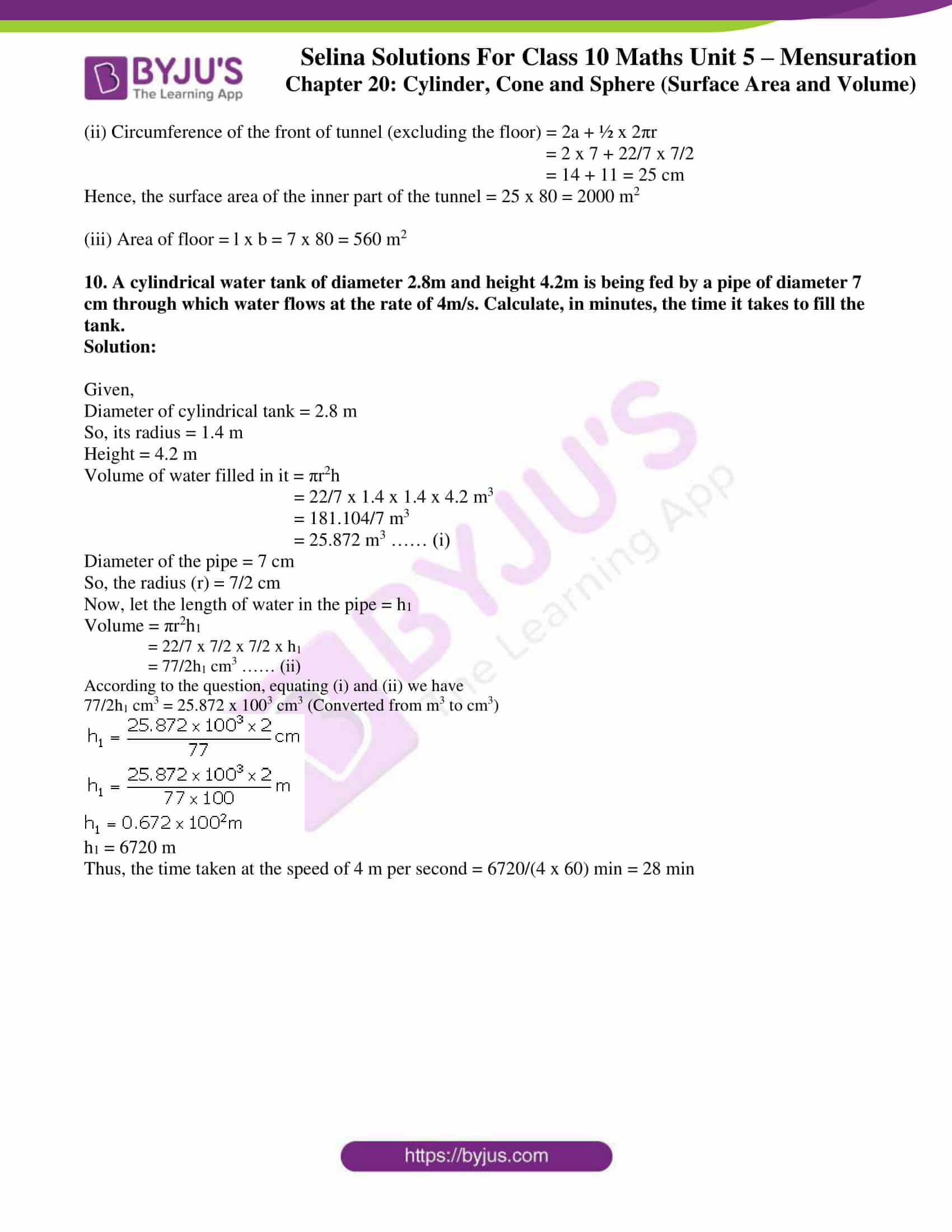### Exercises of Concise Selina Solutions Class 10 Maths Chapter 20 Cylinder, Cone and Sphere (Surface Area and Volume)

Exercise 20(A) Solutions

Exercise 20(B) Solutions

Exercise 20(C) Solutions

Exercise 20(D) Solutions

Exercise 20(E) Solutions

Exercise 20(F) Solutions

Exercise 20(G) Solutions

## Access Selina Solutions Concise Maths Class 10 Chapter 20 Cylinder, Cone and Sphere (Surface Area and Volume)

Exercise 20(A) Page No: 297

1. The height of a circular cylinder is 20 cm and the radius of its base is 7 cm. Find:

(i) the volume

(ii) the total surface area.

Solution:

Given, a circular cylinder whose

Height, h = 20 cm and base radius, r = 7 cm

(i) Volume of cylinder = πr2h = 22/7 x 72 x 20 cm3

= 3080 cm3

(ii) Total surface area of a cylinder = 2πr (h + r)

= 2 x 22/7 x 7(20 + 7) cm2

= 2 x 22 x 27 cm3

= 1188 cm3

2. The inner radius of a pipe is 2.1 cm. How much water can 12 m of this pipe hold?

Solution:

Given,

The inner radius of the pipe = 2.1 cm

Length of the pipe = 12 m = 1200 cm

Volume of the pipe = πr2h = 22/7 x 2.12 x 1200

= 16632 cm3

3. A cylinder of circumference 8 cm and length 21 cm rolls without sliding for 4½ seconds at the rate of 9 complete rounds per second. Find:

(i) distance travelled by the cylinder in 4½ seconds, and

(ii) the area covered by the cylinder in 4½ seconds

Solution: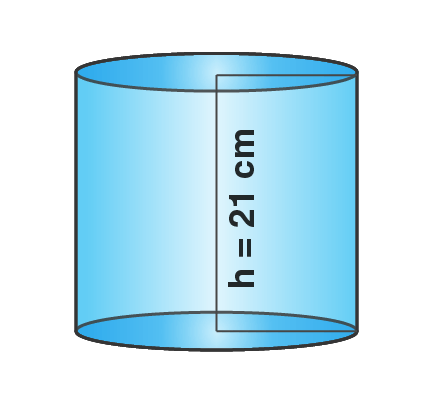Given,

Base circumference of cylinder (c) = 8 cm

So, the radius = c/2π = (8 x 7)/ (2 x 22) = 14/11 cm

Length of the cylinder (h) = 21 cm

(i) If distance covered in one revolution is 8 cm, then distance covered in 9 revolutions = 9 x 8 = 72 cm or distance covered in 1 second = 72 cm.

Thus, distance covered in 4½ seconds = 72 x (9/2) = 324 cm

(ii) Curved surface area = 2πrh

= 2 x 22/7 x 14/11 x 21

= 168 cm2

So, the area covered in one revolution = 168 cm2

Then,

The area covered in 9 revolutions = 168 x 9 = 1512 cm2

Which is also the area covered in 1 second = 1512 cm2

Therefore, the area covered in 4½ seconds = 1512 x 9/2 = 6804 cm2

4. How many cubic meters of earth must be dug out to make a well 28 m deep and 2.8 m in diameter? Also, find the cost of plastering its inner surface at Rs 4.50 per sq meter.

Solution:

Given,

Radius of the well = 2.8/2 = 1.4 m

Depth of the well = 28 m

Hence, the volume of earth dug out = πr2h

= (22/7) x 1.4 x 1.4 x 28

= 17248/100 = 172.48 m3

Area of curved surface = 2πrh

= 2 x 22/7 x 1.4 x 28

= 246.40 m2

Now, the cost of plastering at the rate of Rs 4.50 per sq m

= Rs 246.40 x 4.50

= Rs 1108.80

5. What length of solid cylinder 2 cm in diameter must be taken to recast into a hollow cylinder of external diameter 20 cm, 0.25 cm thick and 15 cm long?

Solution:

Given,

External diameter of hollow cylinder = 20 cm

So, it’s radius = 10 cm = R

Thickness = 0.25 cm

Hence, the internal radius = (10 – 0.25) = 9.75 cm = r

Length of cylinder (h) = 15 cm

Now,

Volume = πh (R2 – r2) = π x 15(102 – 9.752) = 15 π (100 – 95.0625)

= 15 π x 4.9375 cm3

Now,

Diameter of the solid cylinder = 2 cm

so, radius (r) = 1 cm

Let h be the length of the solid cylinder,

Volume = πr2h = π12h = πh cm3

Then, according to given condition in the question

πh = 15 π x 4.9375

h = 15 x 4.9375

h = 74.0625

Thus, the length of solid cylinder = 74.0625 cm

6. A cylinder has a diameter of 20 cm. The area of curved surface is 100 sq cm. Find:

(i) the height of the cylinder correct to one decimal place.

(ii) the volume of the cylinder correct to one decimal place.

Solution:

Given,

The diameter of the cylinder = 20 cm

So, the radius (r) = 10 cm

And the curved surface area = 100 cm2

Height = h cm

(i) Curved surface area = 2πrh

So,

2πrh = 100 cm2

2 x 22/7 x 10 x h = 100

h = (100 x 7)/ (22 x 10 x 2) = 35/22

h = 1.6 cm

(ii) Volume of the cylinder = πr2h

= 22/7 x 10 x 10 x 1.6

= 502.9 cm3

7. A metal pipe has a bore (inner diameter) of 5 cm. The pipe is 5 mm thick all round. Find the weight, in kilogram, of 2 metres of the pipe if 1 cm3 of the metal weights 7.7 g.

Solution:

Given,

Inner radius of the pipe = r = 5/2 = 2.5 cm

External radius of the pipe = R = Inner radius of the pipe + Thickness of the pipe

= 2.5 cm + 0.5 cm = 3 cm

Length of the pipe = h = 2m = 200 cm

Volume of the pipe = External Volume – Internal Volume

= πR2h – πr2h

= π(R2 – r2)h

= π (R + r)(R – r) h

= 22/7 (3 – 2.5)(3 + 2.5) x 200

= 22/7 (0.5) (5.5) x 200 = 1728.6 cm3

Since, 1 cm3 of the metal weight 7.7g,

Hence, weight of the pipe = (1728.6 x 7.7)g = (1728.6 x 7.7)/ 1000 kg = 13.31 kg

8. A cylindrical container with diameter of base 42 cm contains sufficient water to submerge a rectangular solid of iron with dimensions 22 cm x 14 cm x 10.5 cm. Find the rise in level of the water when the solid is submerged.

Solution:

Given,

Diameter of cylindrical container = 42 cm

So, it’s radius (r) = 21 cm

Dimensions of rectangular solid = 22cm x 14cm x 10.5cm

Hence,

The volume of solid = 22 x 14 x 10.5 cm3 ….. (i)

Let the height of water = h

So, the volume of water in the container will be = πr2h

= 22/7 x 21 x 21 x h cm3 …… (ii)

According to the question, from (i) and (ii) we have

22/7 x 21 x 21 x h = 22 x 14 x 10.5

22 x 3 x 21 x h = 22 x 14 x 10.5

h = (22 x 14 x 10.5)/ (22 x 3 x 21)

= 7/3 = 2.33 cm

Therefore, the water level will be raised to a level of 2.33 cm when the solid is submerged.

9. A cylindrical container with internal radius of its base 10 cm, contains water up to a height of 7 cm. Find the area of wetted surface of the cylinder.

Solution:

Given,

Internal radius of the cylindrical container = 10 cm = r

Height of water = 7 cm = h

So, the surface area of the wetted surface = 2πrh + πr2 = πr(2h + r) = 22/7 x 10 x (2 x 7 + 10)

= 220/7 x 24

= 754.29 cm2

10. Find the total surface area of an open pipe of length 50 cm, external diameter 20 cm and internal diameter 6 cm.

Solution:

Given,

Length of the open pipe = 50 cm

Its external diameter = 20 cm

So, it’s external radius (R) = 10 cm

And, its internal diameter = 6 cm

So, it’s internal radius (r) = 3 cm

Then.

Surface area of pipe open from both sides = 2πRh + 2πrh = 2πh(R + r)

= 2 x 22/7 x 50 x (10 + 3)

= 4085.71 cm2

Area of upper and lower part = 2π(R2 – r2)

= 2 x 22/7 x (102 – 32)

= 2 x 22/7 x 91

= 572 cm2

Hence, the total surface area = 4085.71 + 572 = 4657.71 cm3

11. The height and the radius of the base of a cylinder are in the ratio 3:1. If its volume is 1029π cm3; find its total surface area.

Solution:

Given,

The ratio between height and radius of a cylinder = 3:1

Volume = 1029π cm3  …….(i)

Let the radius of the base = r

Then, it’s height will be = 3r

Hence,

Volume = πr2h = π x r2 x 3r = 3πr3 …… (ii)

Equating (i) and (ii), we get

3πr3 = 1029π

r3 = 1029π/ 3π

r3 = 343

r = 7

Thus, radius = 7 cm and height = 3 x 7 = 21 cm

Now,

Total surface area = 2πr(h + r) = 2 x 22/7 x 7 x (21 + 7)

= 2 x 22/7 x 7 x 28

= 44 x 28

= 1232 cm2

12. The radius of a solid right circular cylinder increases by 20% and its height decreases by 20%. Find the percentage change in its volume.

Solution:

Let the radius of a solid right cylinder (r) = 100 cm

And, let the height of a solid right circular cylinder (h) = 100 cm

So, the volume (original) of a solid right circular cylinder = πr2h

= π x (100)2 x 100

= 10000 π cm3

Now, the new radius = r’ = 120 cm

New height = h’ = 80 cm

So, the volume (new) of a solid right circular cylinder = πr’2h’ = π x (120)2 x 80

= 1152000 π cm3

Thus, the increase in volume = New volume – Original volume

= 1152000 π cm3 – 1000000 π cm

= 152000 π cm3

Therefore, percentage change in volume = Increase in volume/Original volume x 100%

= 152000 π cm3/ 1000000 π cm3 x 100%

= 15.2 %

13. The radius of a solid right circular cylinder decreases by 20% and its height increases by 10%. Find the percentage change in its:

(i) volume (ii) curved surface area

Solution:

Let the original dimensions of the solid right cylinder be radius (r) and height (h) in cm.

Then its volume = πr2h cm3

And, curved surface area = 2πrh

Now, after the changes the new dimensions are:

New radius (r’) = r – 0.2r = 0.8r and

New height (h’) = h + 0.1h = 1.1h

So,

The new volume = πr’2h’ cm3

= π(0.8r)2(1.1h) cm3

= 0.704 πr2h cm3

And, the new curved surface area = 2πr’h’ = 2π(0.8r)(1.1h)

= (0.88) 2πrh

(i) Percentage change in its volume = Decrease in volume/ original volume x 100 %

= (Original volume – new volume)/ original volume x 100 %

= (πr2h – 0.704 πr2h)/ πr2h x 100

= 0.296 x 100 = 29.6 %

(ii) Percentage change in its curved surface area = Decrease in CSA/ original CSA x 100 %

= (Original CSA – new CSA)/ original CSA x 100 %

= (2πrh – (0.88) 2πrh)/ 2πrh x 100

= 0.12 x 100 = 12 %

14. Find the minimum length in cm and correct to nearest whole number of the thin metal sheet required to make a hollow and closed cylindrical box of diameter 20 cm and height 35 cm. Given that the width of the metal sheet is 1 m. Also, find the cost of the sheet at the rate of Rs. 56 per m.

Find the area of metal sheet required, if 10% of it is wasted in cutting, overlapping, etc.

Solution:

Given,

Height of the cylinder box = h = 35 cm

Base radius of the cylinder box = r = 10 cm

Width of metal sheet = 1m = 100 cm

Area of metal sheet required = total surface area of the box

Length x width = 2πr(r + h)

Length x 100 = 2 x 22/7 x 10(10 + 35)

Length x 100 = 22 x 22/7 x 10 x 45

Length = (2 x 22 x 10 x 45)/ (100 x 7) = 28.28 cm = 28 cm (correcting to the nearest whole number)

Thus,

Area of metal sheet = length x width = 28 x 100 = 2800 cm2 = 0.28 m2

So, the cost of the sheet at the rate of Rs 56 per m2 = Rs (56 x 0.28) = Rs 15.68

Let the total sheet required be x.

Then, x – 10% of x = 2800 cm2

x – 10/100 × x = 2800 cm2

9x = 2800

x = 3111 cm2

Therefore, a metal sheet of area 3111 cm2 is required.

Exercise 20(B) Page No: 303

1. Find the volume of a cone whose slant height is 17 cm and radius of base is 8 cm.

Solution:

Given,

Slant height of the cone (l) = 17 cm

Base radius (r) = 8 cm

We know that,

l2 = r2 + h2

h2 = l2 – r2 = 172 – 82 = 289 – 64 = 225

h = 15 cm

Now, the volume of cone = 1/3 πr2h = 1/3 x 22/7 x 8 x 8 x 15 cm3

= 7040/7 cm3

= 1005.71 cm3

2. The curved surface area of a cone is 12320 cm2. If the radius of its base is 56 cm, find its height.

Solution:

Given,

Curved surface area of the cone = 12320 cm2

Radius of the base = 56 cm

Let the slant height be ‘l’

Then,

Curved surface area = πrl = 12320 cm2

22/7 x 56 x l = 12320 cm2

l = (12320 x 7)/ (22 x 56)

l = 70 cm

We know that,

l2 = h2 + r2

702 = h2 + 562

h2 = 4900 – 3136 = 1764

Thus, h = 42 cm

3. The circumference of the base of a 12 m high conical tent is 66 m. Find the volume of the air contained in it.

Solution:

Given,

Circumference of the base (c) = 66 m

Height of the conical tent (h) = 12 m

Radius = c/2π = 66/ 2π = (33 x 7)/22 = 21/2 = 10.5 m

Thus, the volume of the cone tent = 1/3 πr2h

= 1/3 x 22/7 x (21/2)2 x 12

= 1386 m3

Therefore, the volume of air contained is 1386 m3.

4. The radius and height of a right circular cone are in the ratio 5:12 and its volume is 2512 cubic cm. Find the radius and slant height of the cone. (Take π = 3.14)

Solution: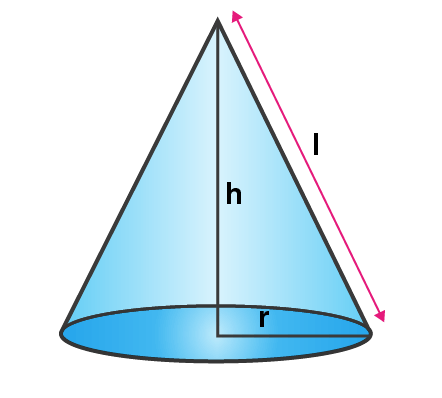Given,

The ratio between radius and height = 5: 12

Volume of the right circular cone = 2512 cm3

Let its radius (r) = 5x, its height (h) = 12x and slant height = l

We know that,

l2 = r2 + h2 = (5x)2 + (12x)2 = 25x2 + 144x2

l2 = 169x2

l = 13x

Now, the volume = 1/3 πr2 h

1/3 πr2 h = 2512

1/3 x (3.14) x (5x)2 x (12x) = 2512

1/3 x (3.14) x (300x3) = 2512

x3 = (2512 x 3)/ (3.14 x 300) = 8

x = 2

Thus,

Radius = 5x = 5 x 2 = 10 cm

Height = 12x = 12 x 2 = 24 cm

Slant height = 13x = 13 x 2 = 26 cm

5. Two right circular cones x and y are made, x having three times the radius of y and y having half the volume of x. Calculate the ratio between the heights of x and y.

Solution:From the question,

Let radius of cone y = r

So, radius of cone x = 3r

Let volume of cone y = V

Then, volume of cone x = 2V

Let h1 be the height of x and h2 be the height of y.

Now,

Volume of cone x = 1/3 π(3r)2h1 = 1/3 π9r2h1 = 3πr2h1

Volume of cone y = 1/3 πr2h2

So,

2V/V = 3πr2h1/ (1/3 πr2h2)

2 = 3h1 x 3/ h2 = 9h1/ h2

h1/h2 = 2/9

Hence, h1: h2 = 2: 9

6. The diameters of two cones are equal. If their slant heights are in the ratio 5:4, find the ratio of their curved surface areas.

Solution:

Let radius of each cone = r

Given that, ratio between their slant heights = 5: 4

Let slant height of the first cone = 5x

And slant height of second cone = 4x

So, curved surface area of the first cone = πrl = πr x (5x) = 5πrx

And, the curved surface area of the second cone = πr x (4x) = 4πrx

Therefore,

The ratio between them = 5πrx: 4πrx = 5: 4

7. There are two cones. The curved surface area of one is twice that of the other. The slant height of the latter is twice that of the former. Find the ratio of their radii.

Solution:

Let slant height of the first cone = l

So, the slant height of the second cone = 2l

Radius of the first cone = r1

And, the radius of the second cone = r2

Now,

Curved surface area of first cone = πr1l

Curved surface area of second cone = πr2(2l) = 2πr2l

According to given condition, we have

πr1l = 2(2πr2l)

r1 = 4r2

r1/ r2 = 4/1

Thus, r1: r2 = 4: 1

Exercise 20(C) Page No: 305

1. The surface area of a sphere is 2464 cm2, find its volume.

Solution:

Given,

Surface area of the sphere = 2464 cm2

Let the radius of the sphere be r.

Then, surface area of the sphere = 4πr2

4πr2 = 2464

4 x 22/7 x r2 = 2464

r2 = (2464 x 7)/ (4 x 22) = 196

r = 14 cm

So, volume = 4/3 πr3

= 4/3 x 22/7 x 14 x 14 x 14

= 11498.67 cm3

2. The volume of a sphere is 38808 cm3; find its diameter and the surface area.

Solution:

Given,

Volume of the sphere = 38808 cm3

Let the radius of the sphere = r

4/3 πr3 = 38808

4/3 x (22/7) x r3 = 38808

r3 = (38808 x 7 x 3)/ (4 x 22) = 9261

r = 21 cm

So, the diameter = 2r = 21 x 2 = 42 cm

And,

Surface area = 4πr2 = 4 x 22/7 x 21 x 21 cm2 = 5544 cm2

3. A spherical ball of lead has been melted and made into identical smaller balls with radius equal to half the radius of the original one. How many such balls can be made?

Solution:

Let the radius of the spherical ball = r

So, the volume = 4/3 πr3

And, the radius of smaller ball = r/2

Volume of smaller ball = 4/3 π(r/2)3 = 4/3 πr3/8 = πr3/6

Thus, the number of balls made out of the given ball = (4/3 πr3)/ (πr3/6)

= 4/3 x 6 = 8

4. How many balls each of radius 1 cm can be made by melting a bigger ball whose diameter is 8 cm.

Solution:

Given,

Diameter of bigger ball = 8 cm

So, radius of bigger ball = 4 cm

Volume = 4/3 πr3 = 4/3 π 43 = 265π/3 cm3

Radius of small ball = 1 cm

Then, volume = 4/3 πr3 = 4/3 π 13 = 4π/3 cm3

Thus,

The number of balls = (265π/3)/ (4π/3)

= 256π/3 x 3/4π

= 64

5. 8 metallic sphere; each of radius 2 mm, are melted and cast into a single sphere. Calculate the radius of the new sphere.

Solution:

Radius of metallic sphere = 2mm = 1/5 cm

Volume = 4/3 πr3 = 4/3 x 22/7 x 1/5 x 1/5 x 1/5 = 88/ (21 x 125) cm3

Volume of 8 spheres = (88 x 8)/ (21 x 125) = 704/ (21 x 125) cm3 ….. (i)

Let the radius of new sphere = R

Volume = 4/3 πR3 = 4/3 x 22/7 x R3 …… (ii)

According the question, equating (i) and (ii) we have

704/ (21 x 125) = 4/3 x 22/7 x R3

704/ (21 x 125) = 88/21 x R3

R3 = (704 x 21)/ (21 x 125 x 88) = 8/125

R = 2/5 = 0.4 cm = 4 mm

Therefore, the radius of the new sphere is 4 mm.

6. The volume of one sphere is 27 times that of another sphere. Calculate the ratio of their:

(ii) surface areas

Solution:

Given,

The volume of first sphere = 27 x volume of second sphere

Let the radius of the first sphere = r1

And, radius of second sphere = r2

(i) Then, according to the question we have

4/3 πr13 = 27 (4/3 πr23)

r13/ r23 = 27

r1/ r2 = 3/ 1

Thus, r1: r2 = 3: 1

(ii) Surface area of the first sphere = 4 πr12

And the surface area of second sphere = 4 πr22

Ratio of their surface areas = 4 πr12/ 4 πr22 = r12/ r22 = 32/ 12 = 9

Hence, the ratio = 9: 1

Exercise 20(D) Page No: 308

1. A solid sphere of radius 15 cm is melted and recast into solid right circular cones of radius 2.5 cm and height 8 cm. Calculate the number of cones recast.

Solution:

Given,

Radius of the sphere = R = 15 cm

So, the volume of sphere melted = 4/3 πR2 = 4/3 x π x 15 x 15 x 15

Radius of each cone recasted = r = 2.5 cm

And, height of each cone recasted = h = 8 cm

So, volume of each cone recasted = 1/3 πr2h = 1/3 x π x 2.5 x 2.5 x 8

Thus,

Number of cones recasted = Volume of sphere melted/ Volume of each cone formed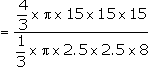= 270

2. A hollow sphere of internal and external diameters 4 cm and 8 cm respectively is melted into a cone of base diameter 8 cm. Find the height of the cone.

Solution:

Given,

External diameter of the hollow sphere = 8 cm

So, radius (R) = 4 cm

Internal diameter of the hollow sphere = 4 cm

So, radius (r) = 2 cm

Then, the volume of metal used in hollow sphere =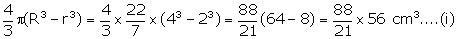Also given,

Diameter of cone = 8 cm

Let height of the cone = h

Volume = 1//3 πr2h = 1/3 x 22/7 x 4 x 4 x h = 352/21 h …. (ii)

So, according to the question

From (i) and (ii), we haveh = (88 x 56 x 21)/ (21 x 352) = 14 cm

Thus, the height of the cone = 14 cm

3. The radii of the internal and external surfaces of a metallic spherical shell are 3 cm and 5 cm respectively. It is melted and recast into a solid right circular cone of height 32 cm. find the diameter of the base of the cone.

Solution:

Given,

Height of the solid right circular cone = 32 cm

Internal radius metallic spherical shell = 3 cm

External radius spherical shell = 5 cm

Then, the volume of the spherical shell = 4/3 π (53 – 33)

= 4/3 x 22/7 x (125 – 27)

= 4/3 x 22/7 x 98

Volume of solid right circular cone = 1/3 πr2h

= 1/3 x 22/7 x r2 x 32

According to the question,

1/3 x 22/7 x r2 x 32 = 4/3 x 22/7 x 98

r2 = (4 x 98)/ 32

r = 7/2 = 3.5 cm

Therefore, diameter = 2r = 7 cm

4. Total volume of three identical cones is the same as that of a bigger cone whose height is 9 cm and diameter 40 cm. Find the radius of the base of each smaller cone, if height of each is 108 cm.

Solution:

Let the radius of the smaller cone be r cm

Given,

Diameter of bigger cone = 40 cm

So, the radius = 20 cm and height = 9 cm

Volume of larger cone = 1/3 π x (20)2 x 9

And,

The volume of the smaller cone = 1/3 π x r2 x 108

From the question, we have

Volume of larger cone = 3 x Volume of smaller cone

1/3 π x (20)2 x 9 = 3 x (1/3 π x r2 x 108)

r2 = (20)2 x 9/ (108 x 3)

r = 20/6 = 10/3 cm

5. A solid rectangular block of metal 49 cm by 44 cm by 18 cm is melted and formed into a solid sphere. Calculate the radius of the sphere.

Solution:

Volume of rectangular block = 49 x 44 x 18 cm3 = 38808 cm3 …… (i)

Let r be the radius of sphere.

So, the volume = 4/3 πr3 = 4/3 x 22/7 x r3 = 88/21 r3 ….. (ii)

Then, according to the question

88/21 r3 = 38808

r3 = (38808 x 21)/ 88 = 441 x 21

= 9261

r = 21 cm

Therefore, the radius of sphere = 21 cm

6. A hemi-spherical bowl of internal radius 9 cm is full of liquid. This liquid is to be filled into conical shaped small containers each of diameter 3 cm and height 4 cm. How many containers are necessary to empty the bowl?

Solution:

Given,

Radius of hemispherical bowl = 9 cm

Volume = 2/3 πr3 = 2/3 π93 = 2/3 π x 729 = 486 π cm2

Diameter of each cylindrical bottle = 3 cm

So, the radius = 1.5 cm and height = 4 cm

Volume of bottle = 1/3 πr2h = 1/3 π(3/2)2 x 4 = 3 π

Thus,

The number of bottles = 486 π/ 3 π

= 162

Exercise 20(E) Page No: 311

1. A cone of height 15 cm and diameter 7 cm is mounted on a hemisphere of same diameter. Determine the volume of the solid thus formed.

Solution: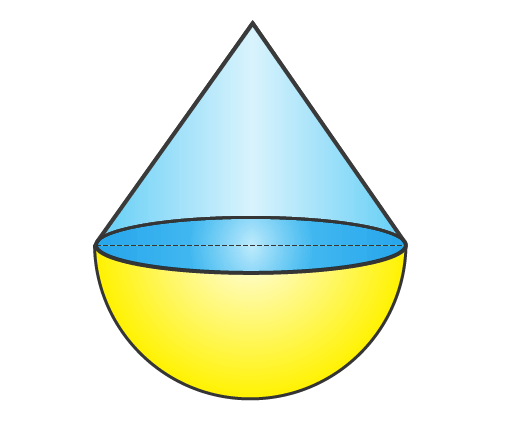Given,

Height of the cone = 15 cm

Diameter of the cone = 7 cm

So, its radius = 3.5 cm

Radius of the hemisphere = 3.5 cm

Now,

Volume of the solid = Volume of the cone + Volume of the hemisphere

= 1/3 πr2 h + 2/3 πr3

= 1/3 x 22/7 x (3.5)2 x 15 + 2/3 x 22/7 x (3.5)3

= 1/3 x 22/7 x (3.5)2 (15 + 2×3.5)

= 847/3

= 282.33 cm3

2. A buoy is made in the form of a hemisphere surmounted by a right cone whose circular base coincides with the plane surface of the hemisphere. The radius of the base of the cone is 3.5 m and its volume is two-third of the hemisphere. Calculate the height of the cone and the surface area of the buoy, correct to two decimal places.

Solution:

Given,

Radius of the hemisphere part (r) = 3.5 m = 7/2 m

Volume of hemisphere = 2/3 πr3

= 2/3 x 22/7 x 7/2 x 7/2 x 7/2

= 539/6 m3

Volume of conical part = 2/3 x 539/6 m3 [2/3 rd of the hemisphere]

Let height of the cone = h

Then,

1/3 πr2 h = (2 x 539)/ (3 x 6)

1/3 x 22/7 x 7/2 x 7/2 x h = (2 x 539)/ (3 x 6)

h = (2 x 539 x 2 x 2 x 7 x 3)/ (3 x 6 x 22 x 7 x 7)

h = 14/3 m = 4.67 m

Height of the cone = 4.67 m

Surface area of buoy = 2 πr2 + πrl

But, we know that

l = (r2 + h2)1/2

l = ((7/2)2 + (14/3)2)1/2

l = (1225/36)1/2 = 35/6 m

Hence,

Surface area = 2πr2 + πrl

= (2 x 22/7 x 7/2 x 7/2) + (22/7 x 7/2 x 35/6)

= 77 + 385/6 = 847/6

= 141.17 m2 [Corrected to 2 decimal places]

3. From a rectangular solid of metal 42 cm by 30 cm by 20 cm, a conical cavity of diameter 14 cm and depth 24 cm is drilled out. Find:

(i) the surface area of the remaining solid

(ii)the volume of remaining solid

(iii) the weight of the material drilled out if it weighs 7 gm per cm3.

Solution: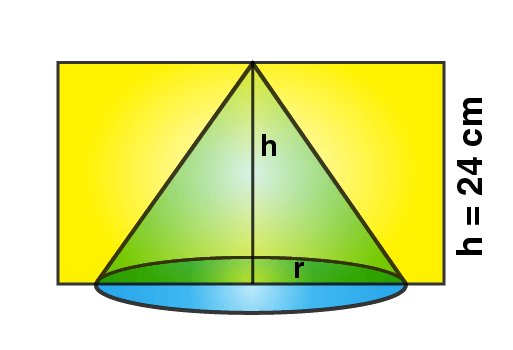Given,

Dimensions of rectangular solid l = 42 cm, b = 30 cm and h = 20 cm

Conical cavity’s diameter = 14 cm

So, its radius = 7 cm

Depth (height) = 24 cm

(i) Total surface area of cuboid = 2 (lb + bh + lh)

= 2 (42 x 30 + 30 x 20 + 20 x 42)

= 2 (1260 + 600 + 840)

= 2 (2700)

= 5400 cm2

Diameter of the cone = 14 cm

So, its radius = 14/2 = 7 cm

Area of circular base = πr2 = 22/7 x 7 x 7 = 154 cm2

We know that,

l = (72 + 242)1/2 = (49 + 576)1/2 = (625)1/2 = 25

Area of curved surface area of cone = πrl = 22/7 x 7 x 25 = 22 x 25 = 550 cm2

Surface area of remaining part = 5400 + 550 – 154 = 5796 cm2

(ii) Volume of the rectangular solid = (42 x 30 x 20) cm3 = 25200 cm3

Radius of conical cavity (r) = 7 cm

Height (h) = 24 cm

Volume of cone = 1/3 πr2 h

= 1/3 x 22/7 x 7 x 7 x 24

= 1232 cm3

(iii) Weight of material drilled out = 1232 x 7g = 8624g = 8.624 kg

4. The cubical block of side 7 cm is surmounted by a hemisphere of the largest size. Find the surface area of the resulting solid.

Solution:

It’s known that, the diameter of the largest hemisphere that can be placed on a face of a cube of side 7 cm will be 7 cm.

So, it’s radius = r = 7/2 cm

It’s curved surface area = 2 πr2 = 2 x 22/7 x 7/2 x 7/2 = 77 cm2 …… (i)

Now,

Surface area of the top of the resulting solid = Surface area of the top face of the cube – Area of the base of the hemisphereSurface area of the cube = 5 x (side)2 = 5 x 49 = 245 cm2 …… (iii)

Hence,

Total area of resulting solid = (i) + (ii) + (iii) = 245 + 10.5 + 77 = 332.5 cm2

5. A vessel is in the form of an inverted cone. Its height is 8 cm and the radius of the top which is open is 5 cm. It is filled with water up to the rim. When lead shots, each of which is a sphere of radius 0.5 cm, are dropped into the vessel, one-fourth of the water flows out. Find the number of lead shots dropped in the vessel.

Solution: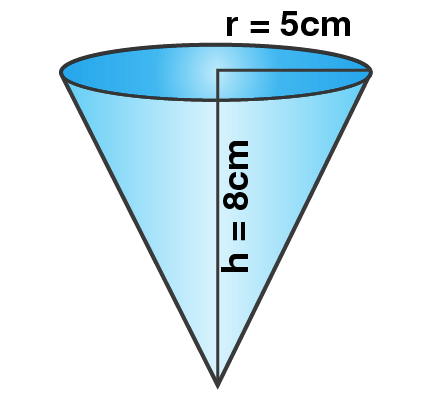Given,

Height of cone = 8 cm

Volume = 1/3 πr2 h

= 1/3 x 22/7 x 5 x 5 x 8 cm3

= 4400/21 cm3

Hence, the volume of water that flowed out = ¼ x 4400/21 cm3

= 1100/21 cm3

Also, given

Radius of each ball = 0.5 cm = ½ cm

Volume of a ball = 4/3 πr3

= 4/3 x 22/7 x ½ x ½ x ½ cm3

= 11/21 cm3

Thus, the no. of balls = 1100/21 = 11/21 = 100

Exercise 20(F) Page No: 315

1. From a solid right circular cylinder with height 10 cm and radius of the base 6 cm, a right circular cone of the same height and same base are removed. Find the volume of the remaining solid.

Solution:

Given,

Height of the cylinder (h) = 10 cm

And radius of the base (r) = 6 cm

Volume of the cylinder = πr2 h

Height of the cone = 10 cm

Radius of the base of cone = 6 cm

Volume of the cone = 1/3 πr2 h

Volume of the remaining part = πr2 h – 1/3 πr2 h

= 2/3 πr2 h

= 2/3 x 22/7 x 6 x 6 x 10

= 5280/7 cm3

= 754 (2/7) cm3

2. From a solid cylinder whose height is 16 cm and radius is 12 cm, a conical cavity of height 8 cm and of base radius 6 cm is hollowed out. Find the volume and total surface area of the remaining solid.

Solution: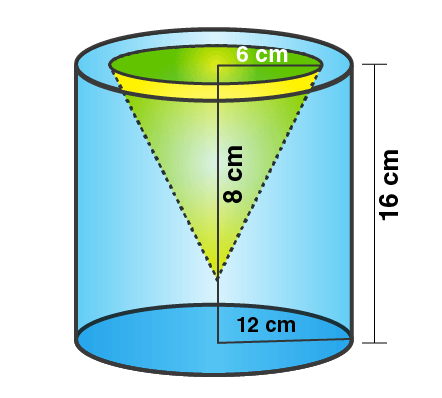Given,

Radius of solid cylinder (R) = 12 cm

And, Height (H) = 16 cm

Volume = πR2 h = 22/7 x 12 x 12 x 16

= 50688/7 cm3

Also given,

Radius of cone (r) = 6 cm, and height (h) = 8 cm.

Volume = 1/3 πr2 h

= 1/3 x 22/7 x 6 x 6 x 8

= 2112/7 cm3

(i) Volume of remaining solid = 50688/7 – 2112/7

= 48567/7

= 6939.43 cm3

(ii) Slant height of cone l = (h2 + r2)1/2

= (62 + 82)1/2 = (36 + 64)1/2 = (100)1/2 = 10 cm

Thus,

Total surface area of remaining solid = curved surface area of cylinder + curved surface area of cone + base area of cylinder + area of circular ring on upper side of cylinder= 15312/7

= 2187.43 cm2

3. A circus tent is cylindrical to a height of 4 m and conical above it. If its diameter is 105 m and its slant height is 80 m, calculate the total area of canvas required. Also, find the total cost of canvas used at Rs 15 per meter if the width is 1.5 m

Solution: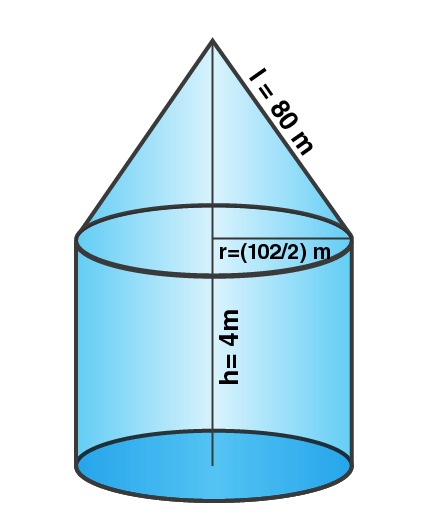Given,

Radius of the cylindrical part of the tent (r) =105/2 m

Slant height (l) = 80 m

So, the total curved surface area of the tent = 2πr h + πrl

= (2 x 22/7 x 105/2 x 4) + (22/7 x 105/2 x 80)

= 1320 + 13200

= 14520 m2

Width of canvas used = 1.5 m

Length of canvas = 14520/1.5 = 9680 m

Hence,

Total cost of canvas at the rate of Rs 15 per meter = 9680 x 15 = Rs 145200

4. A circus tent is cylindrical to a height of 8 m surmounted by a conical part. If total height of the tent is 13 m and the diameter of its base is 24 m; calculate:

(i) total surface area of the tent

(ii) area of canvas, required to make this tent allowing 10% of the canvas used for folds and stitching.

Solution: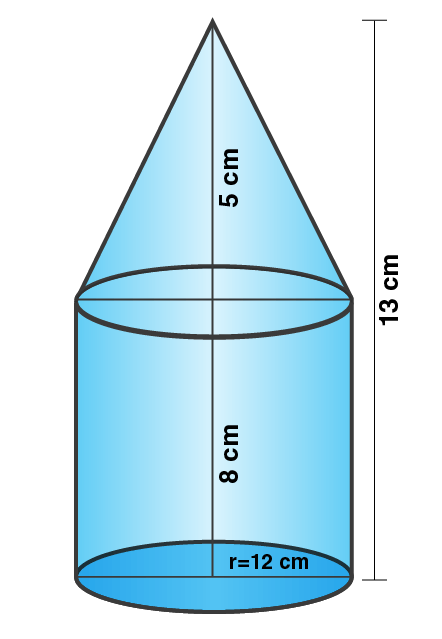Given,

Height of the cylindrical part = H = 8 m

Height of the conical part = h = (13 – 8) m = 5 m

Diameter = 24 m

So, its radius = 24/2 = 12 m

Then, slant height (l) = (r2 + h2)1/2

= (122 + 52)1/2 = (144 + 25)1/2 = (169)1/2 = 13 m

(i) Total surface area of the tent = 2πrh + πrl = πr(2h + l)

= 22/7 x 12 x (2 x 8 + 13)

= 264/7 (16 + 3)

= 264/7 x 29

= 7656/7 m2

= 1093.71 m2

(ii) Area of canvas used in stitching = total area of canvas

Total area of canvas = 7656/7 + total area of canvas/10

Total area of canvas – Total area of canvas/10 = 7656/10

Total area of canvas (1 – 1/10) = 7656/7

Total area of canvas x 9/10 = 7656/7

Total area of canvas = 7656/7 x 10/9 = 76560/63

Therefore, the total area of canvas = 1215.23 m2

5. A cylindrical boiler, 2 m high, is 3.5 m in diameter. It has a hemispherical lid. Find the volume of its interior, including the part covered by the lid.

Solution: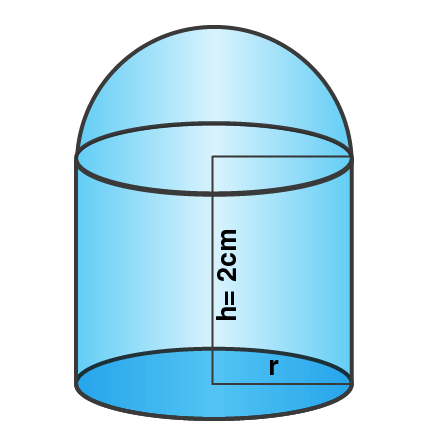Given,

Diameter of cylindrical boiler = 3.5 m

So, the radius (r) = 3.5/2 = 35/20 = 7/4 m

Height (h) = 2m

Radius of hemisphere (R) = 7/4 m

Total volume of the boiler = πr2h + 2/3 πr3

= πr2(h + 2/3r)

= 22/7 x 7/4 x 7/4 (2 + 2/3 x 7/4)

= 77/8 (2 + 7/6)

= 77/8 x 19/6 = 1463/48

= 30.48 m3

6. A vessel is a hollow cylinder fitted with a hemispherical bottom of the same base. The depth of the cylindrical part is 4(2/3) m and the diameter of hemisphere is 3.5 m. Calculate the capacity and the internal surface area of the vessel.

Solution: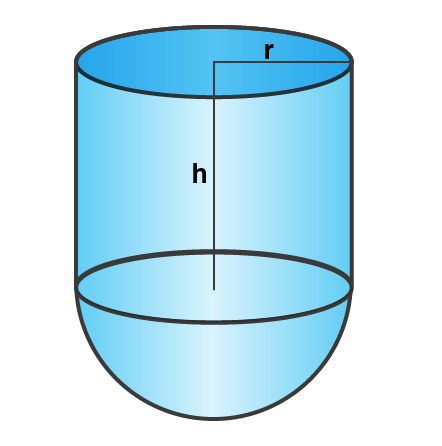Given,

Diameter of the base = 3.5 m

So, its radius = 3.5/2 m = 1.75 m = 7/4 m

Height of cylindrical part = 4 + 2/3 = 14/3

(i) Capacity (volume) of the vessel = πr2h + 2/3 πr2 = πr2 (h + 2/3r)

= 22/7 x 7/4 x 7/4(14/3 + 2/3 x 7/4)

= 77/8(14/3 + 7/6)

= 77/8(28+7/6)

= 77/8 x 35/6

= 2695/48

= 56.15 m3

(ii) Internal curved surface area = 2πrh + 2πr2 = 2πr(h + r)

= 2 x 22/7 x 7/4(14/3 + 7/4)

= 11(56 + 21)/12

= 11 x 77/12

= 847/12

= 70.58 m2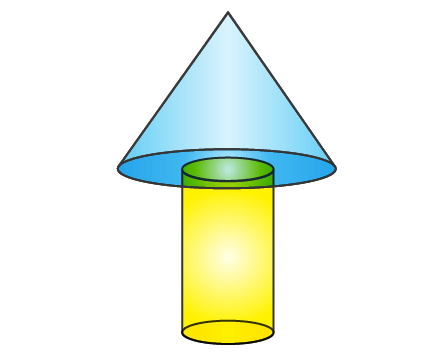7. A wooden toy is in the shape of a cone mounted on a cylinder as shown alongside.

If the height of the cone is 24 cm, the total height of the toy is 60 cm and the radius of the base of the cone = twice the radius of the base of the cylinder = 10 cm; find the total surface area of the toy. [Take π = 3.14]

Solution:

Given,

Height of the cone (h) = 24 cm

Height of the cylinder (H) = 36 cm

Radius of the cone (r) = twice the radius of the cylinder = 10 cm

Radius of the cylinder (R) = 5 cm

Then, we know the slant height of the cone = (r2 + h2)1/2 = (102 + 242)1/2 = (100 + 576)1/2

= (676)1/2 = 26 cm

Now, the surface area of the toy = curved area of the conical point + curved area of the cylinder

= πrl + πr2 + 2πRH

= π (rl + r2 + 2RH)

= 3.14 (10 x 26 + 102 + 2x5x36)

= 3.14 (260 + 100 + 360)

= 3.14 (720)

= 2260.8 cm2

Exercise 20(G) Page No: 316

1. What is the least number of solid metallic spheres, each of 6 cm diameter, that should be melted and recast to form a solid metal cone whose height is 45 cm and diameter is 12 cm?

Solution:

Given,

Diameter of solid metallic sphere = 6 cm

So, its radius = 3 cm

Height of solid metal cone = 45

Diameter of metal cone = 12 cm

So, its radius = 6 cm

Now,

Let the number of solid metallic spheres be ‘n’

Volume of 1 sphere = 4/3 π (3)3

Volume of metallic cone = 1/3 π62 x 45

Hence, n = Volume of metal cone/ Volume of 1 sphere

n = (1/3 π62 x 45)/ (4/3 π (3)3)

= (6 x 6 x 45)/ (4 x 3 x 3 x 3)

n = 15

2. A largest sphere is to be carved out of a right circular cylinder of radius 7 cm and height 14 cm. Find the volume of the sphere. (Answer correct to the nearest integer)

Solution:

Radius of the largest sphere that can be formed inside the cylinder will be equal to the radius of the cylinder.

So, radius of the largest sphere = 7 cm

Volume of sphere = 4/3 π 73

= 4/3 x 22/7 x 7 x 7 x 7

= 4312/3

= 1437 cm3

3. A right circular cylinder having diameter 12 cm and height 15 cm is full of ice-cream. The ice-cream is to be filled in identical cones of height 12 cm and diameter 6 cm having a hemi-spherical shape on the top. Find the number of cones required.

Solution:

Given,

Diameter of the cylinder = 12 cm

So, its radius = 6 cm

Height of the cylinder = 15 cm

Diameter of the cone = 6 cm

So, its radius = 3 cm

Height of the cone = 12 cm

Radius of the hemisphere = 3 cm

Now,

Let the number of cones be ‘n’.

Volume of the cylinder = πr2h = π 62 x 15

Volume of an ice-cream cone with ice-cream = Volume of cone + Volume of hemisphere

= 1/3 π(3)2 x 12 + 2/3 π(3)2

= 36 π + 18 π

= 54 π cm3

Number of cones = Volume of cylinder/ Volume of ice-cream cone

= π 62 x 15 / 54 π

= (36 x 15)/ 54

= 10

Therefore, the number of cones required = 10

4. A solid is in the form of a cone standing on a hemisphere with both their radii being equal to 8 cm and the height of cone is equal to its radius. Find in terms of πthe volume of the solid.

Solution:

Given,

Radius of both cone and hemisphere = 8 cm

And, height = 8 cm

Hence,

Volume of the solid = Volume of cone + volume of hemisphere

= 1/3 πr2h + 2/3 πr3

= 1/3 π(8)2(8) + 2/3 π(8)3

= π(8)3

= 512 π cm3

5. The diameter of a sphere is 6 cm. It is melted and drawn into a wire of diameter 0.2 cm. Find the length of wire.

Solution:

Given,

Diameter of the sphere = 6 cm

So, its radius = 3 cm

And, volume = 4/3 πr3 = 4/3 x 22/7 x 3 x 3 x 3 = 792/7 cm3 ….. (i)

Diameter of cylindrical wire = 0.2 cm

So, the radius of the wire = 0.2/2 = 0.1 = 1/10 cm

Now, let length of wire = h

Volume = πr2 h = 22/7 x 1/10 x 1/10 x h cm3 = 22h/700 cm3 ….. (ii)

According to the question, equating (i) and (ii) we have

22h/700 = 792/7

h = 792/7 x 700/22

h = 3600 cm = 36 m

Therefore, length of the wire = 36 m

6. Determine the ratio of the volume of a cube to that of a sphere which will exactly fit inside the cube.

Solution:

Let edge of the cube = a

Then, volume of the cube = a x a x a = a3

The sphere that exactly fits in the cube will have radius ‘a/2’

So,

Volume of sphere = 4/3 πr3 = 4/3 x 22/7 x (a/2)3 = 4/3 x 22/7 x a3/8 = 11/21 a3

Now,

Volume of cube: Volume of sphere

= a3 : 11/21 a3

= 1 : 11/21

= 21 : 11

7. An iron pole consisting of a cylindrical portion 110 cm high and of base diameter 12 cm is surmounted by a cone 9 cm high. Find the mass of the pole, given that 1 cm3 of iron has 8 gm of mass (approx). (Take π = 355/113)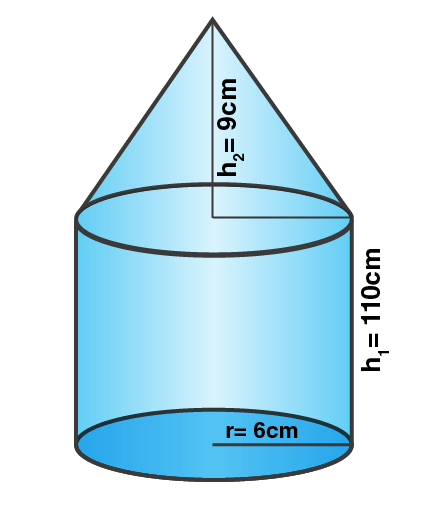Solution:

Given,

Radius of the base of poles (r) = 6 cm

Height of the cylindrical part (h1) = 110 cm

Height of the conical part (h2) = 9 cm

Now,

Total volume of the iron pole = πr2h1 + 1/3 πr2h2 = πr2 (h1 + 1/3h2)

= 355/113 x 6 x 6(110 + 1/3 x 9)

= 355/113 x 36 x 113

= 12780 cm3

Weight of 1 cm3 = 8 gm (Given)

Thus, the total weight = 12780 x 8 = 102240 gm = 102.24 kg

8. In the following diagram a rectangular platform with a semicircular end on one side is 22 meters long from one end to the other end. If the length of the half circumference is 11 meters, find the cost of constructing the platform, 1.5 meters high at the rate of Rs 4 per cubic meters.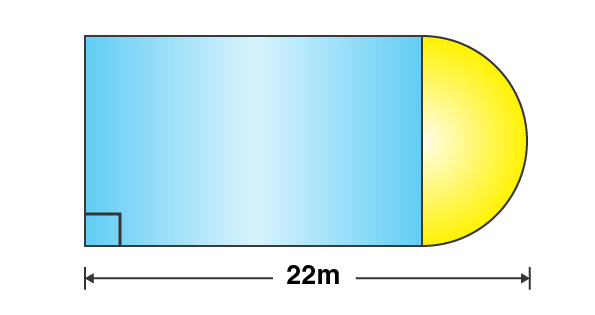Solution:

Given,

Length of the platform = 22 m

Circumference of semi-circle (c) = 11 m

So, its radius = (c x 2)/ (2 x π) = (11 x 7)/ 22 = 7/2 m

Thus, the breadth of the part = 7/2 x 2 = 7 m

And, length = 22 – 7/2 = 37/2 = 18.5 m

Now, area of platform = l x b + ½ πr2

= 18.5 x 7 + ½ x 22/7 x 7/2 x 7/2 m2

= 129.6 + 77/4 m2

= 148.75 m2

Also given, height of the platform = 1.5 m

So, the volume = 148.75 x 1.5 = 223.125 m3

Rate of construction = Rs 4 per m3

Therefore,

Total expenditure = Rs 4 x 223.125 = Rs 892.50

9. The cross-section of a tunnel is a square of side 7 m surmounted by a semicircle as shown in the following figure.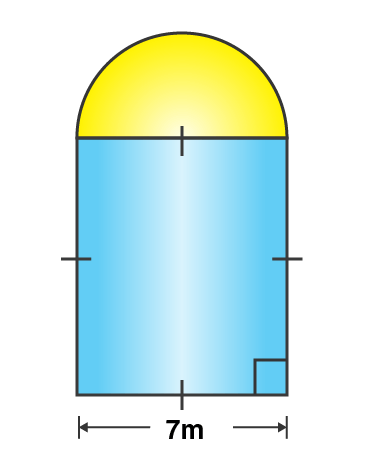The tunnel is 80 m long. Calculate:

(i) its volume

(ii) the surface area of the tunnel (excluding the floor) and

(iii) its floor area

Solution:

Given,

Side of square (a) = 7m

And, the radius of semi-circle = 7/2 m

Length of the tunnel = 80 m

Area of cross section of the front part = a2 + ½ πr2

= 7 x 7 + ½ x 22/7 x 7/2 x 7/2

= 49 + 77/4 m2

= (196 + 77)/ 4

= 273/4 m2

(i) Thus, the volume of the turnel = area of cross section x length of the turnel

= 273/4 x 80

= 5460 m3

(ii) Circumference of the front of tunnel (excluding the floor) = 2a + ½ x 2πr

= 2 x 7 + 22/7 x 7/2

= 14 + 11 = 25 cm

Hence, the surface area of the inner part of the tunnel = 25 x 80 = 2000 m2

(iii) Area of floor = l x b = 7 x 80 = 560 m2

10. A cylindrical water tank of diameter 2.8m and height 4.2m is being fed by a pipe of diameter 7 cm through which water flows at the rate of 4m/s. Calculate, in minutes, the time it takes to fill the tank.

Solution:

Given,

Diameter of cylindrical tank = 2.8 m

So, its radius = 1.4 m

Height = 4.2 m

Volume of water filled in it = πr2h

= 22/7 x 1.4 x 1.4 x 4.2 m3

= 181.104/7 m3

= 25.872 m3 …… (i)

Diameter of the pipe = 7 cm

So, the radius (r) = 7/2 cm

Now, let the length of water in the pipe = h1

Volume = πr2h1

= 22/7 x 7/2 x 7/2 x h1

= 77/2h1 cm3 …… (ii)

According to the question, equating (i) and (ii) we have

77/2h1 cm3 = 25.872 x 1003 cm3 (Converted from m3 to cm3)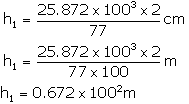h1 = 6720 m

Thus, the time taken at the speed of 4 m per second = 6720/(4 x 60) min = 28 min

The given solutions are as per the 2019-20 Concise Selina textbook. The Selina Solutions for the academic year 2023-24 will be updated soon.